JP2014115431A - Imaging lens, imaging apparatus, and portable terminal - Google Patents

Imaging lens, imaging apparatus, and portable terminal Download PDF

Info

Publication number
JP2014115431A
JP2014115431A JP2012268865A JP2012268865A JP2014115431A JP 2014115431 A JP2014115431 A JP 2014115431A JP 2012268865 A JP2012268865 A JP 2012268865A JP 2012268865 A JP2012268865 A JP 2012268865A JP 2014115431 A JP2014115431 A JP 2014115431A
Authority
JP
Japan
Prior art keywords
lens
imaging lens
imaging
lt
a10
Prior art date
Legal status (The legal status is an assumption and is not a legal conclusion. Google has not performed a legal analysis and makes no representation as to the accuracy of the status listed.)
Pending
Application number
JP2012268865A
Other languages
Japanese (ja)
Inventor
Eigo Sano

Masae Sato

Kiyono Tateyama

Manami Kuiseko

Original Assignee
Konica Minolta Inc
コニカミノルタ株式会社
Priority date (The priority date is an assumption and is not a legal conclusion. Google has not performed a legal analysis and makes no representation as to the accuracy of the date listed.)
Filing date
Publication date
Application filed by Konica Minolta Inc, コニカミノルタ株式会社 filed Critical Konica Minolta Inc
Priority to JP2012268865A priority Critical patent/JP2014115431A/en
Publication of JP2014115431A publication Critical patent/JP2014115431A/en
Application status is Pending legal-status Critical

• 230000004075 alteration Effects 0 abstract 2
• 230000014509 gene expression Effects 0 abstract 2
• 238000003384 imaging method Methods 0 abstract title 8
• 230000001603 reducing Effects 0 abstract 1
• 238000006722 reduction reaction Methods 0 abstract 1
• 230000001629 suppression Effects 0 abstract 1

Images

•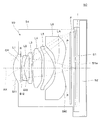••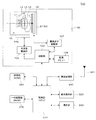•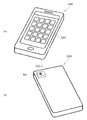•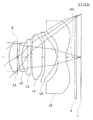•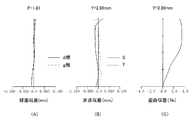•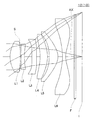•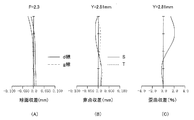•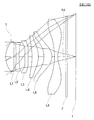•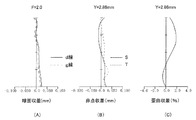•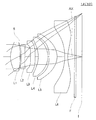•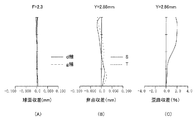•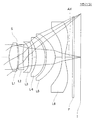•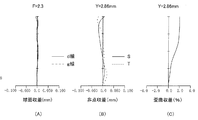•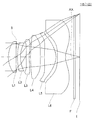•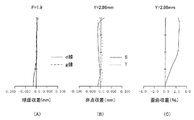•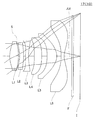•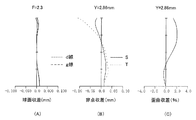•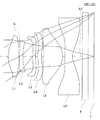•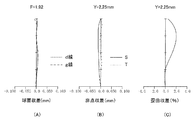•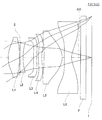•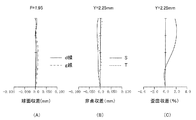•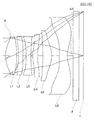•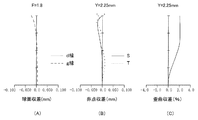•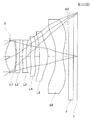•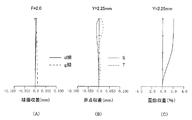•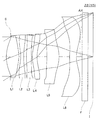•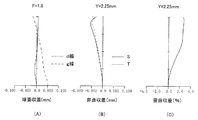•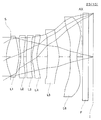•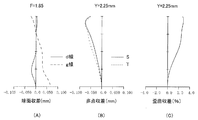Abstract

PROBLEM TO BE SOLVED: To provide an imaging lens which is compact, in which various aberrations are satisfactorily corrected, which has brightness of F2.4 or more, and which has a six lens configuration.SOLUTION: An imaging lens 10 includes, in order from an object side; a positive lens group comprising a first lens L1, a second lens L2, a third lens L3, a fourth lens L4, and a fifth lens L5; and a negative sixth lens L6. The imaging lens 10 satisfies a conditional expression (1): 0.3≤f/(2×f1×Fno)<1.0 ...(1), where f represents a focal length of the entire system of the imaging lens 10, f1 represents a focal length of the first lens L1, and Fno represents an F-number of the entire system of the imaging lens 10. By setting a value below the upper limit of the conditional expression (1), spherical aberration produced in the first lens L1 can be suppressed low. On the other hand, by setting a value above the lower limit, refractive power of the first lens L1 can be appropriately kept, and reduction of the total length of the imaging lens 10 can be achieved. A more preferable range is expressed as: 0.3≤f/(2×f1×Fno)<0.8...(1)'.

Description

The present invention relates to an imaging lens, and an imaging apparatus and a portable terminal including the imaging lens.

In recent years, with the widespread use of portable terminals equipped with an imaging device using an imaging device such as a CCD-type image sensor or a CMOS-type image sensor, an imaging device having a high number of pixels is provided so that a higher quality image can be obtained. Those equipped with built-in imaging devices have been supplied to the market. An image pickup device having a high number of pixels has been accompanied by an increase in size, but in recent years, an increase in the size of pixels has progressed, and an image pickup device having a high number of pixels has been downsized. As the performance of an image pickup device increases and the size of the image pickup device advances, the conventional image pickup lens design method tends to be closer to the so-called telephoto side. However, it is not desirable to approach the telephoto side just because the image sensor has advanced performance and size reduction. In addition, a high resolving power is required for an imaging lens used in such a high-definition image sensor, but the resolving power is limited by the F number, and an F number of about F2.8 as in the past. However, it has become impossible to obtain sufficient performance. Therefore, a bright imaging lens having an F of 2.4 or less, which is suitable for an imaging device with a high pixel size, a high resolution, and a small size, has been demanded. As an imaging lens for such applications, a six-lens imaging lens has been proposed that can have a larger aperture ratio and higher performance than a four- or five-lens configuration.

As a six-lens imaging lens, a first lens having a positive refractive power, a second lens having a negative refractive power, an aperture stop, a third lens having a positive refractive power, and a negative refractive power in order from the object side. An imaging lens including a fourth lens having a fifth lens having a positive refractive power and a sixth lens having a negative refractive power is disclosed (for example, Patent Document 1).

However, the imaging lens described in Patent Document 1 has an aperture stop disposed behind the second lens, and in order to ensure good telecentric characteristics, the entire length of the imaging lens must be increased and the size of the imaging lens can be reduced. Not suitable for.

Further, in a configuration similar to the above-mentioned Patent Document 1, in order from the object side, a first lens having a positive refractive power, a second lens having a negative refractive power, a third lens having a negative refractive power, a positive lens An imaging lens configured by a fourth lens having a refractive power, a fifth lens having a positive refractive power, and a sixth lens having a negative refractive power is disclosed (for example, Patent Document 2).

However, it cannot be said that the imaging lens described in Patent Document 2 is sufficiently small because of insufficient aberration correction. Further, the F number is as dark as F2.8, and it has not been able to cope with the recent increase in pixels and performance.

JP 2012-155223 A US Publication 2012/0188654

The present invention has been made in view of the problems of the background art, and has a six-image structure having a brightness of F2.4 or more with various aberrations corrected satisfactorily while being smaller than the conventional type. The object is to provide a lens.

Here, although it is a scale of a small imaging lens, the present invention aims at miniaturization at a level satisfying the following expression. By satisfying this range, the entire imaging apparatus can be reduced in size and weight.
L / 2Y <1.00 (9)
However,
L: Distance on the optical axis from the lens surface closest to the object side to the image-side focal point of the entire imaging lens system 2Y: Diagonal length of the imaging surface of the imaging device (diagonal length of the rectangular effective pixel region of the solid-state imaging device)
Here, the image-side focal point refers to an image point when a parallel light beam parallel to the optical axis is incident on the imaging lens.
When a parallel plate such as an optical low-pass filter, an infrared cut filter, or a seal glass of the image sensor package is disposed between the image-side surface of the imaging lens and the image-side focal position, the parallel plate The part is assumed to be the air conversion distance and the value of L is calculated.

The value L / 2Y is more preferably in the range of the following formula.
L / 2Y <0.90 (9) ′

In order to achieve the above object, an imaging lens according to the present invention is an imaging lens for forming a subject image on an imaging element, has a brightness of F2.4 or more, and is positive in order from the object side. A first lens having a refractive power and having a convex surface facing the object side in the vicinity of the optical axis, a second lens having a negative refractive power, a third lens, a fourth lens, a fifth lens, and the vicinity of the optical axis And the sixth lens having negative refractive power with the concave surface facing the image side, the image side surface of the sixth lens is aspherical, has an extreme value at a position other than the intersection with the optical axis, and has an aperture stop. Is disposed between the object side surface of the first lens and the image side surface of the second lens, and satisfies the following conditional expression.
0.3 ≦ f / (2 × f1 × Fno) <1.0 (1)
However,
f: focal length of the entire imaging lens f1: focal length of the first lens Fno: F-number of the entire imaging lens

The basic configuration of the imaging lens according to the present invention for obtaining a compact imaging lens with good aberration correction has, in order from the object side, a positive refractive power and a convex surface directed toward the object side in the vicinity of the optical axis. A first lens, a second lens having a negative refractive power, a third lens, a fourth lens, a fifth lens, and a sixth lens having a negative refractive power with a concave surface facing the image side in the vicinity of the optical axis It has become. This lens configuration of a so-called telephoto type in which a positive lens group including a first lens, a second lens, a third lens, a fourth lens, and a fifth lens and a negative sixth lens are arranged in order from the object side. This is an advantageous configuration for reducing the overall length of the imaging lens.

In addition, by using two or more negative lenses in the six-lens configuration, it is possible to easily correct the Petzval sum by increasing the surface having a diverging action, and to secure an excellent imaging performance up to the periphery of the screen. Can be obtained. Furthermore, by making the object side surface of the first lens convex, the combined principal point position of the entire imaging lens system can be moved closer to the object side, which is advantageous in reducing the overall length of the imaging lens.

Further, by making the image side surface of the sixth lens disposed closest to the image side an aspherical surface, various aberrations at the peripheral portion of the screen can be corrected satisfactorily. Furthermore, the aspherical shape having an extreme value at a position other than the intersection with the optical axis makes it easy to ensure the telecentric characteristics of the image-side light beam. Here, “extreme value” means that the tangent plane or tangent of the aspherical vertex is a plane or line segment perpendicular to the optical axis when considering a curved lens surface or a lens cross-sectional shape within an effective radius. A line or point on a non-spherical surface.

Further, by arranging the aperture stop between the object side surface of the first lens and the image side surface of the second lens, it is possible to achieve both reduction in the overall length of the imaging lens and good telecentric characteristics. Furthermore, by setting the F number of the imaging lens to a brightness of F2.4 or less, it is possible to obtain an imaging lens that can cope with recent high performance. In the above description, the arrangement of the aperture stop between the object side surface of the first lens and the image side surface of the second lens means that the point on the optical axis of the object side surface of the first lens and the light on the image side surface of the second lens. This means an arrangement with respect to a point on the axis.

Conditional expression (1) corresponds to higher brightness in order to cope with higher performance, and further, the F-number is set to a brightness of 2.4 or less. Further, in the six-lens configuration, the focal length of the first lens is the entire imaging lens. As a result of paying attention to the fact that it is one of the factors affecting the F-number, this is a conditional expression for defining the single NA of the first lens, reducing the overall length of the imaging lens, and performing good aberration correction.

First, the meaning of conditional expression (1) will be described. The F-number Fno of the imaging lens is expressed as follows: f is the focal length of the entire imaging lens system, and D is the aperture of the entire imaging lens system.
Fno = f / D
Given in. Here, assuming that the focal length of the entire imaging lens system is normalized to 1 and that the aperture stop is disposed in the vicinity of the first lens, the F number FnoL1 of the first lens unit is set to f1 as the focal length of the first lens. As
FnoL1 = (f1 / f) / (1 / Fno) = f1 / (f × Fno)
It becomes. Since the numerical aperture NA of the optical system is given by NA = 1 / (2 × Fno), the numerical aperture NAL1 of the first lens unit is
NAL1 = 1 / (2 × (f1 / (f × Fno))) = f / (2 × f1 × Fno)
It becomes. That is, the numerical aperture NAL1 of the first lens unit is represented by the approximate value f / (2 × f1 × Fno).

By falling below the upper limit of the conditional expression (1) regarding the numerical aperture NAL1, the NA of the first lens unit does not become too large, and the spherical aberration that occurs in the first lens can be kept small. On the other hand, by exceeding the lower limit of the conditional expression (1), it is possible to appropriately maintain the refractive power of the first lens, and it is possible to reduce the overall length of the imaging lens.
The value f / (2 × f1 × Fno) is more preferably in the range of the following equation.
0.3 ≦ f / (2 × f1 × Fno) <0.8 (1) ′

In a specific aspect of the present invention, in the imaging lens, the following conditional expression (2)
−1.1 <f6 / f <−0.1 (2)
However,
f6: Focal length of the sixth lens f: The focal length of the entire imaging lens system is satisfied.

Conditional expression (2) is a conditional expression for setting the focal length of the sixth lens appropriately so as to achieve both of ensuring the back focus, shortening the total length of the imaging lens, and correcting aberrations. When the value f6 / f of conditional expression (2) exceeds the lower limit, the negative refractive power of the sixth lens does not become too strong, and it becomes easy to secure the back focus, while it is lower than the upper limit of conditional expression (2). Thus, since the negative refractive power of the sixth lens can be appropriately maintained, the overall length of the imaging lens can be shortened, and axial chromatic aberration can be favorably corrected. More preferably, the range of the following formula is good.
The value Tf6 / f is more preferably in the range of the following equation.
−1.0 <f6 / f <−0.2 (2) ′

In another aspect of the present invention, the following conditional expression (3)
−1.8 <f2 / f <−0.1 (3)
However,
f2: Focal length of the second lens f: The focal length of the entire imaging lens system is satisfied.

Conditional expression (3) is a conditional expression for appropriately setting the focal length of the second lens. When the value f2 / f is lower than the upper limit, the negative refractive power of the second lens is not increased more than necessary, and coma and distortion at the peripheral portion can be reduced. On the other hand, exceeding the lower limit of conditional expression (3) makes it possible to maintain the negative refractive power of the second lens appropriately, and is effective in reducing Petzval sum and correcting field curvature. More preferably, the range of the following formula is good.
The value Tf2 / f is more preferably in the range of the following formula.
-1.7 <f2 / f <-0.2 (3) '

In still another aspect of the present invention, the following conditional expression (4)
0.3 <r1 / f <0.6 (4)
However,
r1: radius of curvature of the object side surface of the first lens f: the focal length of the entire imaging lens system is satisfied.

Conditional expression (4) is a conditional expression for appropriately setting the radius of curvature of the object side surface of the first lens to appropriately shorten the imaging lens and to correct the aberration. Considering that the first lens has a relatively strong curvature on the object side surface, the value r1 / f of conditional expression (4) is less than the upper limit, so that the refractive power of the object side surface of the first lens is appropriately maintained. The composite principal point of the first lens and the second lens can be arranged closer to the object side, and the overall length of the imaging lens can be shortened. On the other hand, by exceeding the limit of the conditional expression (4), the refractive power of the object side surface of the first lens is not increased more than necessary, and high-order spherical aberration and coma generated in the first lens are reduced. Can be suppressed.
The value r1 / f is more preferably in the range of the following formula.
0.35 <r1 / f <0.55 (4) ′
Is satisfied.

In still another aspect of the present invention, the following conditional expression (5)
0.02 <THIL2 / f <0.15 (5)
However,
THIL2: Thickness on the optical axis of the second lens f: The focal length of the entire imaging lens system is satisfied.

Conditional expression (5) is a conditional expression for appropriately setting the thickness of the second lens on the optical axis. When the value THIL2 / f of conditional expression (5) exceeds the lower limit, the thickness of the second lens does not become too thin, and the moldability is not impaired. On the other hand, by falling below the upper limit of conditional expression (5), the thickness of the second lens does not become too thick, and it becomes easy to secure the lens interval before and after L2, and as a result, the entire length of the imaging lens can be shortened. More preferably, the range of the following formula is good.
The value THIL2 / f is more preferably in the range of the following formula.
0.03 <THIL2 / f <0.12 (5) '

In still another aspect of the present invention, the following conditional expression (6)
0.03 <THIL6 / f <0.30 (6)
However,
THIL6: Thickness on the optical axis of the sixth lens f: The focal length of the entire imaging lens system is satisfied.

Conditional expression (6) is a conditional expression for appropriately setting the thickness of the sixth lens on the optical axis. When the value THIL6 / f of conditional expression (6) exceeds the lower limit, the thickness of the sixth lens does not become too thin, and the moldability is not impaired. On the other hand, by falling below the upper limit of conditional expression (6), the thickness of the sixth lens does not become too thick, and it becomes easy to ensure the back focus. More preferably, the range of the following formula is good.
0.05 ≦ THIL6 / f <0.25 (6) ′ or 0.10 <THIL6 / f <0.30 (6) ”
Or it can also be set as the range of the following Formula.
0.15 <THIL6 / f <0.25 (6-2)

In still another aspect of the present invention, the second lens has a shape with a concave surface facing the image side. By making the image side surface of the second lens concave, it is possible to give a strong divergence action to the second lens having a relatively high passing ray height, so that field curvature and distortion can be corrected well. It becomes like this.

In still another aspect of the present invention, the following conditional expression (7)
0 ≦ ν5-ν6 <50 (7)
However,
ν5: Abbe number of the fifth lens ν6: The Abbe number of the sixth lens is satisfied.

Conditional expression (7) is a conditional expression for favorably correcting the chromatic aberration of the entire imaging lens system. When the value ν5-ν6 of conditional expression (7) exceeds the lower limit, chromatic aberrations such as axial chromatic aberration and lateral chromatic aberration can be corrected in a well-balanced manner. On the other hand, it can comprise with the easily available glass material by being less than the upper limit of conditional expression (7).
The value ν5-ν6 is more preferably in the range of the following formula.
0 ≦ ν5-ν6 <45 (7) ′

In still another aspect of the present invention, the following conditional expression (8)
20 <ν1-ν2 <70 (8)
However,
ν1: Abbe number of the first lens ν2: The Abbe number of the second lens is satisfied.

Conditional expression (8) is a conditional expression for satisfactorily correcting the chromatic aberration of the entire imaging lens system. When the value ν1−ν2 of the conditional expression (8) exceeds the lower limit, chromatic aberration such as axial chromatic aberration and lateral chromatic aberration can be corrected in a well-balanced manner. On the other hand, it can comprise with the easily available glass material by being less than the upper limit of conditional expression (8).
The value ν1-ν2 is more preferably in the range of the following formula.
25 <ν1-ν2 <65 (8) ′

In still another aspect of the present invention, the first lens has a meniscus shape. Thus, since the first lens has a meniscus shape, the combined principal point position of the entire imaging lens system can be moved closer to the object side, so that the overall length of the imaging lens can be shortened. .

In still another aspect of the present invention, the lens further includes a lens having substantially no power.

In order to achieve the above object, an imaging apparatus according to the present invention includes the imaging lens described above and an imaging element. By using the imaging lens of the present invention, it is possible to obtain an imaging apparatus that has a brightness of F2.4 or more and is small and has various aberrations corrected satisfactorily.

In order to achieve the above object, a portable terminal according to the present invention includes an imaging device having a brightness of F2.4 or more and having a small size and excellent correction of various aberrations as described above.

It is a figure explaining an imaging device provided with the imaging lens of one embodiment of the present invention. It is sectional drawing explaining the state of the imaging lens etc. which were hold | maintained in the lens-barrel part. It is a block diagram explaining a portable communication terminal provided with the imaging device of FIG. (A) And (B) is a perspective view of the surface side and back surface side of a portable communication terminal, respectively. 3 is a cross-sectional view of the imaging lens of Example 1. FIG. (A)-(C) are the aberrational figures of the imaging lens of Example 1. FIG. 6 is a cross-sectional view of an imaging lens of Example 2. FIG. (A)-(C) are the aberrational figures of the imaging lens of Example 2. FIGS. 6 is a cross-sectional view of an imaging lens of Example 3. FIG. (A)-(C) are the aberrational figures of the imaging lens of Example 3. FIG. 6 is a cross-sectional view of an imaging lens of Example 4. FIG. (A)-(C) are the aberrational figures of the imaging lens of Example 4. FIG. FIG. 10 is a cross-sectional view of the imaging lens of Example 5. (A)-(C) are the aberrational figures of the imaging lens of Example 5. FIG. 6 is a cross-sectional view of an imaging lens of Example 6. FIG. (A)-(C) are the aberrational figures of the imaging lens of Example 6. FIG. 10 is a cross-sectional view of an imaging lens of Example 7. FIG. (A)-(C) are the aberrational figures of the imaging lens of Example 7. FIG. 10 is a cross-sectional view of an imaging lens of Example 8. FIG. (A)-(C) are the aberrational figures of the imaging lens of Example 8. FIG. 10 is a cross-sectional view of an imaging lens of Example 9. FIG. FIGS. 9A to 9C are aberration diagrams of the imaging lens of Example 9. FIGS. 10 is a cross-sectional view of an imaging lens of Example 10. FIG. FIGS. 9A to 9C are aberration diagrams of the imaging lens of Example 10. FIGS. 14 is a cross-sectional view of the imaging lens of Example 11. FIG. FIGS. 9A to 9C are aberration diagrams of the imaging lens of Example 11. FIGS. 14 is a cross-sectional view of an imaging lens of Example 12. FIG. (A)-(C) are the aberrational figures of the imaging lens of Example 12. FIG. 14 is a cross-sectional view of the imaging lens of Example 13. FIG. FIGS. 9A to 9C are aberration diagrams of the imaging lens of Example 13. FIGS.

Hereinafter, with reference to FIG. 1 etc., the imaging lens which is one Embodiment of this invention is demonstrated. The imaging lens 10 illustrated in FIG. 1 has the same configuration as the imaging lens 11 of Example 1 described later.

FIG. 1 is a cross-sectional view illustrating a camera module including an imaging lens according to an embodiment of the present invention.

The camera module 50 includes an imaging lens 10 that forms a subject image, an imaging device 51 that detects a subject image formed by the imaging lens 10, and a wiring board 52 that holds the imaging device 51 from behind and has wiring and the like. And a lens barrel portion 54 having an opening OP for holding the imaging lens 10 and the like and allowing a light beam from the object side to enter. The imaging lens 10 has a function of forming a subject image on the image plane or the imaging plane (projected plane) I of the imaging element 51. The camera module 50 is used by being incorporated in an imaging device to be described later.

The imaging lens 10 includes, in order from the object side, a first lens L1, a second lens L2, a third lens L3, a fourth lens L4, a fifth lens L5, and a sixth lens L6. The aperture stop S is disposed between the object side surface S11 of the first lens L1 and the image side surface S22 of the second lens L2. The imaging lens 10 is small in size, and as a scale, aims at miniaturization at a level satisfying the following expression (12).
L / 2Y <1.00 (9)
Here, L is the distance on the optical axis from the most object-side lens surface S11 of the entire imaging lens 10 system to the image-side focal point, and 2Y is the diagonal length of the imaging surface of the imaging device 51 (the rectangular effective pixel of the imaging device 51). The image side focal point refers to an image point when parallel light rays parallel to the optical axis AX are incident on the imaging lens 10. By satisfying this range, the entire camera module 50 can be reduced in size and weight.

In the case where a parallel flat plate F such as an optical low-pass filter, an infrared cut filter, or a seal glass of an image pickup device package is disposed between the most image side surface S52 of the image pickup lens 10 and the image side focal position. The parallel plate F portion is calculated as the above L value after the air conversion distance. More preferably, it is in the range of the following formula.
L / 2Y <0.90 (9) ′

The image sensor 51 is a sensor chip made of a solid-state image sensor. The photoelectric conversion unit 51a of the image pickup device 51 includes a CCD (charge coupled device) or a CMOS (complementary metal oxide semiconductor), photoelectrically converts incident light for each RGB, and outputs an analog signal thereof. . The surface of the photoelectric conversion unit 51a as the light receiving unit is an image plane or an imaging plane (projected plane) I.

The wiring board 52 has a role of aligning and fixing the image sensor 51 to other members (for example, the lens barrel portion 54). The wiring board 52 can receive a voltage and a signal for driving the image pickup device 51 and the driving mechanism 55a from an external circuit, and can output a detection signal to the external circuit.

On the imaging lens 10 side of the imaging element 51, a parallel plate F is disposed and fixed by a holder member (not shown) so as to cover the imaging element 51 and the like.

The lens barrel 54 houses and holds the imaging lens 10. The lens barrel portion 54 enables the focusing operation of the imaging lens 10 by moving any one or more of the lenses L1 to L5 constituting the imaging lens 10 along the optical axis AX. For example, it has a drive mechanism 55a. The drive mechanism 55a reciprocates a specific lens along the optical axis AX. The drive mechanism 55a includes, for example, a voice coil motor and a guide. The drive mechanism 55a can be configured by a stepping motor or the like instead of the voice coil motor or the like.

FIG. 2 is a cross-sectional view for explaining the state of the imaging lens 10 and the like held in the lens barrel portion 54. The first to sixth lenses L <b> 1 to L <b> 6 constituting the imaging lens 10 each have a supporting flange portion 39, and are joined to adjacent lenses via the flange portion 39. Between these lenses L <b> 1 to L <b> 6, light shielding plates 31, 32, 33, 34, and 35 are disposed so as to be sandwiched by the flange portion 39. Any one of the light shielding plates 31 is an aperture stop S that defines the brightness of the imaging lens 10. The light shielding plates 32, 33, 34, and 35 other than the aperture stop S are for preventing the generation of stray light.

Next, an example of a mobile phone or other mobile communication terminal 300 equipped with the camera module 50 illustrated in FIG. 1 will be described with reference to FIGS. 3, 4 (A), and 4 (B).

The mobile communication terminal 300 is a smartphone-type mobile communication terminal or mobile terminal, and the image capturing apparatus 100 having the camera module 50 and a control unit (CPU) that performs overall control of each unit and executes a program corresponding to each process. 310, a display operation unit 320 that is a touch panel that displays data related to communication, captured video, and the like and receives a user operation, an operation unit 330 including a power switch, an external server, and the like via an antenna 341 A wireless communication unit 340 for realizing various information communication between the mobile station and a storage unit (ROM) storing necessary data such as a system program, various processing programs, and a terminal ID of the mobile communication terminal (mobile terminal) 300 360, various processing programs and data executed by the control unit 310, processing data, Includes a temporary storage unit (RAM) 370 used as a work area for temporarily storing the imaging data and the like by the imaging device 100.

In addition to the camera module 50 described above, the imaging apparatus 100 includes a control unit 103, an optical system driving unit 105, an imaging element driving unit 107, an image memory 108, and the like.

The control unit 103 controls each unit of the imaging device 100. The control unit 103 includes a CPU (Central Processing Unit), a RAM (Random Access Memory), a ROM (Read Only Memory), and the like, and various types of programs are read out from the ROM and expanded in the RAM, in cooperation with the CPU. Execute the process. Note that the control unit 310 is communicably connected to the control unit 104 of the imaging apparatus 100, and can exchange control signals and image data.

The optical system driving unit 105 controls the state of the imaging lens 10 by operating the first and second driving mechanisms 55 a of the imaging lens 10 when performing focusing, exposure, and the like under the control of the control unit 103. The optical system driving unit 105 operates the driving mechanism 55a to appropriately move a specific lens in the imaging lens 10 along the optical axis AX, thereby causing the imaging lens 10 to perform a focusing operation.

The image sensor drive unit 107 controls the operation of the image sensor 51 when performing exposure or the like under the control of the control unit 103. Specifically, the image sensor drive unit 107 controls the image sensor 51 by scanning and driving based on the timing signal. Further, the image sensor driving unit 107 converts the detection signal output from the image sensor 51 or an analog signal as a photoelectric conversion signal into digital image data. Further, the image sensor driving unit 107 can perform various image processing such as distortion correction, color correction, and compression on the image signal detected by the image sensor 51.

The image memory 108 receives the digitized image signal from the image sensor driving unit 107 and stores it as readable and writable image data.

Here, the photographing operation of the mobile communication terminal 300 including the imaging device 100 will be described. When the camera mode in which the mobile communication terminal 300 is operated as a camera is set, subject monitoring (through image display) and image shooting execution are performed. In monitoring, an image of a subject obtained through the imaging lens 10 is formed on the imaging surface I (see FIG. 1) of the imaging element 51. The image sensor 51 is scanned and driven by the image sensor driving unit 107, and outputs an analog signal for one screen as a photoelectric conversion output corresponding to a light image formed at regular intervals.

This analog signal is converted into digital data after gain adjustment is appropriately performed for each primary color component of RGB in a circuit attached to the image sensor 51. The digital data is subjected to color process processing including pixel interpolation processing and Y correction processing, and a digital luminance signal Y and color difference signals Cb, Cr (image data) are generated and stored in the image memory 108. The stored digital data is periodically read out from the image memory 108 to generate a video signal thereof, and is output to the display operation unit 320 via the control unit 103 and the control unit 310.

The display operation unit 320 functions as a finder in monitoring and displays captured images in real time. In this state, focusing, exposure, and the like of the imaging lens 10 are set by driving the optical system driving unit 105 based on an operation input performed by the user via the display operation unit 320 at any time.

In such a monitoring state, when the user appropriately operates the display operation unit 320, still image data is captured. One frame of image data stored in the image memory 108 is read in accordance with the operation content of the display operation unit 320, and compressed by the image sensor driving unit 107. The compressed image data is recorded in the RAM 370, for example, via the control unit 103 and the control unit 310.

The above-described imaging apparatus 100 is an example of an imaging apparatus suitable for the present invention, and the present invention is not limited to this.

That is, the image pickup apparatus equipped with the camera module 50 or the image pickup lens 10 is not limited to the one built in the smartphone type mobile communication terminal 300, but is built into a mobile phone, a PHS (Personal Handyphone System), or the like. Alternatively, it may be incorporated in a PDA (Personal Digital Assistant), a tablet personal computer, a mobile personal computer, a digital still camera, a video camera, or the like.

Hereinafter, returning to FIG. 1, the imaging lens 10 according to an embodiment of the present invention will be described in detail. The imaging lens 10 shown in FIG. 1 forms a subject image on the imaging surface (projected surface) I of the imaging device 51, has a brightness of F2.4 or higher, and sequentially increases in order from the object side. A first lens L1, an aperture stop S, a second lens L2 having a negative refractive power, a third lens L3, and a fourth lens L4. And the fifth lens L5 and the sixth lens L6 with the concave surface facing the image side in the vicinity of the optical axis AX. In the imaging lens 10, the image side surface S62 of the sixth lens L6 has an aspheric shape, has an extreme value at a position P other than the intersection with the optical axis AX, and the aperture stop S extends from the object side surface S11 of the first lens L1. Between the two lens L2 and the image side surface S22, in the illustrated example, it is disposed adjacent to the outer edge of the image side surface S12 of the first lens L1. The imaging lens 10 satisfies the conditional expression (1) already described.
0.3 ≦ f / (2 × f1 × Fno) <1.0 (1)
Here, f is the focal length of the entire imaging lens 10, f1 is the focal length of the first lens L1, and Fno is the F number of the entire imaging lens 10.

The imaging lens 10 of the present embodiment includes, in order from the object side, a positive lens group including a first lens L1, a second lens L2, a third lens L3, a fourth lens L4, and a fifth lens L5, and a negative sixth lens. This is a so-called telephoto type configuration in which the lens L6 is disposed. The telephoto type configuration is advantageous for reducing the overall length of the imaging lens.

In addition, by using two or more of the six-lens imaging lens 10 as negative lenses, the number of surfaces having a diverging action is increased to facilitate correction of Petzval sum, and good imaging performance is ensured up to the periphery of the screen. The obtained imaging lens 10 can be obtained. Furthermore, by making the object side surface S11 of the first lens L1 convex, the combined principal point position of the entire imaging lens 10 system can be moved closer to the object side, which is advantageous for reducing the overall length of the imaging lens 10.

In addition, by making the image side surface S62 of the sixth lens L6 disposed closest to the image side an aspherical surface, various aberrations at the peripheral portion of the screen can be favorably corrected. Furthermore, the aspherical shape having an extreme value at a position P other than the intersection with the optical axis AX makes it easy to ensure the telecentric characteristics of the image-side light beam. Here, the “extreme value” is a point on the aspheric surface where the tangent plane of the aspheric vertex is a plane perpendicular to the optical axis AX in the curve of the lens cross-sectional shape within the effective radius.

In addition, by arranging the aperture stop S between the object side surface S11 of the first lens L1 and the image side surface S22 of the second lens L2, it is possible to achieve both a reduction in the overall length of the imaging lens 10 and good telecentric characteristics. it can. Furthermore, by setting the F number of the imaging lens 10 to a brightness of F2.4 or less, it is possible to obtain the imaging lens 10 that can cope with recent high performance.

Conditional expression (1) defines the single NA of the first lens L1, and is a conditional expression for reducing the overall length of the imaging lens 10 and performing good aberration correction. Note that the value f / (2 × f1 × Fno) in the conditional expression (1) corresponds to the numerical aperture of the first lens L1 alone as described above.

By falling below the upper limit of conditional expression (1), the NA of the first lens L1 alone does not become too large, and the spherical aberration that occurs in the first lens L1 can be kept small. On the other hand, by exceeding the lower limit, the refractive power of the first lens L1 can be maintained moderately, and downsizing of the entire length of the imaging lens 10 can be achieved. More preferably, the range of the following formula is good.
0.3 ≦ f / (2 × f1 × Fno) <0.8 (1) ′

In the imaging lens 10 of the embodiment, in addition to the conditional expression (1), the conditional expression (2) already described.
−1.1 <f6 / f <−0.1 (2)
Satisfied. Here, f6 is the focal length of the sixth lens L6, and f is the focal length of the entire imaging lens 10.
More preferably, the imaging lens 10 of the embodiment satisfies the following conditional expression (2) ′.
−1.0 <f6 / f <−0.2 (2) ′

In the imaging lens 10 of the embodiment, in addition to the conditional expressions (1) and (2), the conditional expression (3) already described.
−1.8 <f2 / f <−0.1 (3)
Satisfied. However, f2 is a focal distance of the 2nd lens L2, and f is a focal distance of the imaging lens 10 whole system.
The imaging lens 10 of the embodiment more preferably satisfies the following conditional expression (3) ′.
-1.7 <f2 / f <-0.2 (3) '

In the imaging lens 10 of the embodiment, in addition to the conditional expressions (1) to (3), the conditional expression (4) already described.
0.3 <r1 / f <0.6 (4)
Satisfied. Here, r1 is the radius of curvature of the object side surface S11 of the first lens L1, and f is the focal length of the entire imaging lens 10.
More preferably, the imaging lens 10 of the embodiment satisfies the following conditional expression (4) ′.
0.35 <r1 / f <0.55 (4) ′

In the imaging lens 10 of the embodiment, in addition to the conditional expressions (1) to (4), the conditional expression (5) already described.
0.02 <THIL2 / f <0.15 (5)
Satisfied. Here, THIL2 is the thickness of the second lens L2 on the optical axis AX, and f is the focal length of the entire imaging lens 10 system.
The imaging lens 10 of the embodiment preferably satisfies the following conditional expression (5) ′.
0.03 <THIL2 / f <0.12 (5) '

In the imaging lens 10 of the embodiment, in addition to the conditional expressions (1) to (5), the conditional expression (6) already described.
0.03 <THIL6 / f <0.30 (6)
Satisfied. Here, THIL6 is the thickness of the sixth lens L6 on the optical axis AX, and f is the focal length of the entire imaging lens 10.
More preferably, the imaging lens 10 of the embodiment satisfies the following conditional expression (6) ′ or (6) ″.
0.05 ≦ THIL6 / f <0.25 (6) ′
0.10 <THIL6 / f <0.30 (6) "
Or it can also satisfy | fill the following Formula.
0.15 <THIL6 / f <0.25 (6-2)

In the imaging lens 10 of the embodiment, in addition to the conditional expressions (1) to (6), the conditional expression (7) already described.
0 ≦ ν5-ν6 <50 (7)
Satisfied. Here, ν5 is the Abbe number of the fifth lens L5, and ν6 is the Abbe number of the sixth lens L6.
The imaging lens 10 of the embodiment preferably satisfies the following conditional expression (7) ′.
0 ≦ ν5-ν6 <45 (7) ′

In the imaging lens 10 of the embodiment, in addition to the conditional expressions (1) to (7), the conditional expression (8) already described.
20 <ν1-ν2 <70 (8)
Satisfied. Here, ν1 is the Abbe number of the first lens L1, and ν2 is the Abbe number of the second lens L2.
The imaging lens 10 of the embodiment preferably satisfies the following conditional expression (8) ′.
25 <ν1-ν2 <65 (8) ′

The imaging lens 10 of the embodiment further includes a lens having substantially no power.

The imaging apparatus 100 according to the embodiment includes the imaging lens 10 described above and an imaging element 51.

The mobile communication terminal (mobile terminal) 300 according to the embodiment includes an imaging device that is small and has various aberrations corrected satisfactorily as described above.

〔Example〕
Examples of the imaging lens of the present invention will be shown below. Symbols used in each example are as follows.
f: Focal length of the entire imaging lens system fB: Back focus F: F number 2Y: Diagonal length of the imaging surface of the imaging device ENTP: Entrance pupil position (distance from the first surface to the entrance pupil position)
EXTP: exit pupil position (distance from imaging surface to exit pupil position)
H1: Front principal point position (distance from first surface to front principal point position)
H2: Rear principal point position (distance from the final surface to the rear principal point position)
R: radius of curvature d: axial top surface spacing Nd: refractive index νd of lens material with respect to d-line: Abbe number of lens material In each example, the surface described with “*” after each surface number has an aspherical shape. The aspherical surface shape is expressed by the following “Equation 1”, where the vertex of the surface is the origin, the X axis is taken in the optical axis direction, and the height in the direction perpendicular to the optical axis is h.
However,
Ai: i-order aspheric coefficient R: radius of curvature K: conic constant

Example 1
The overall specifications of the imaging lens of Example 1 are shown below.
f = 3.47mm
fB = 0.29mm
F = 1.81
2Y = 5.7mm
ENTP = 0.4mm
EXTP = -2.5mm
H1 = -0.44mm
H2 = -3.18mm

The lens surface data of Example 1 is shown in Table 1 below.
[Table 1]
Surface number R (mm) d (mm) Nd νd Effective radius (mm)
1 * 1.719 0.55 1.54470 56.2 1.05
2 (STOP) -24.146 0.09 0.90
3 * 4.510 0.20 1.63470 23.9 0.93
4 * 1.771 0.37 0.94
5 * 4.427 0.20 1.63470 23.9 0.98
6 * 2.777 0.09 1.18
7 * 3.617 0.68 1.54470 56.2 1.44
8 * 6.274 0.19 1.49
9 * 132.081 0.78 1.54470 56.2 1.61
10 * -0.790 0.09 1.65
11 * -4.971 0.52 1.54470 56.2 1.73
12 * 0.928 0.60 2.41
13 infinite 0.11 1.51630 64.1 2.72
14 infinite 2.75

The aspheric coefficients of the lens surfaces of Example 1 are shown in Table 2 below.
[Table 2]
First side
K = -0.14072E + 01, A4 = 0.38929E-01, A6 = 0.11304E-01, A8 = 0.15016E-01,
A10 = -0.58592E-01, A12 = 0.75804E-01, A14 = -0.34646E-01
Second side
K = 0.50000E + 02, A4 = 0.78856E-01, A6 = -0.13149E-01, A8 = -0.16713E + 00,
A10 = 0.36760E + 00, A12 = -0.34516E + 00, A14 = 0.11532E + 00
Third side
K = -0.47956E + 02, A4 = 0.53604E-01, A6 = 0.25727E-01, A8 = -0.11048E + 00,
A10 = 0.12403E + 00, A12 = -0.53290E-01
4th page
K = -0.82498E + 01, A4 = 0.79973E-01, A6 = 0.13324E-01, A8 = -0.38437E-01,
A10 = 0.38815E-01
5th page
K = 0.16769E + 02, A4 = -0.22231E + 00, A6 = 0.18631E + 00, A8 = -0.38232E + 00,
A10 = 0.31551E + 00, A12 = -0.15211E + 00
6th page
K = -0.25636E + 02, A4 = -0.16643E + 00, A6 = 0.27573E + 00, A8 = -0.37975E + 00,
A10 = 0.31971E + 00, A12 = -0.15613E + 00, A14 = 0.32579E-01
7th page
K = -0.50000E + 02, A4 = -0.12109E + 00, A6 = 0.13641E + 00, A8 = -0.55292E-01,
A10 = 0.43084E-02, A12 = 0.30013E-02, A14 = -0.46902E-03
8th page
K = 0.0, A4 = -0.10875E + 00, A6 = -0.74101E-02, A8 = -0.13541E-01,
A10 = 0.19732E-01, A12 = -0.11943E-01, A14 = 0.30788E-02
9th page
K = 0.50000E + 02, A4 = 0.23826E-02, A6 = -0.39241E-01, A8 = -0.37907E-01,
A10 = 0.38522E-01, A12 = -0.10795E-01, A14 = 0.11212E-02
10th page
K = -0.40370E + 01, A4 = -0.40310E-01, A6 = -0.13923E-01, A8 = -0.37676E-02,
A10 = 0.45008E-02, A12 = 0.16133E-02, A14 = -0.53944E-03
11th page
K = 0.68701E + 01, A4 = 0.71583E-01, A6 = -0.24897E + 00, A8 = 0.19277E + 00,
A10 = -0.73475E-01, A12 = 0.13043E-01, A14 = -0.67098E-03
12th page
K = -0.68557E + 01, A4 = -0.70387E-01, A6 = 0.21581E-01, A8 = -0.55183E-02,
A10 = 0.72807E-03, A12 = -0.38086E-04, A14 = -0.30918E-06
In the following (including the lens data in the table), a power of 10 (for example, 2.5 × 10 −02 ) is expressed using E (for example, 2.5E-02).

The single lens data of Example 1 is shown in Table 3 below.
[Table 3]
Lens Start surface Focal length (mm)
1 1 2.968
2 3 -4.728
3 5 -12.325
4 7 14.377
5 9 1.444
6 11 -1.392

FIG. 5 is a cross-sectional view of the imaging lens 11 and the like of the first embodiment. The imaging lens 11 includes a biconvex first lens L1 having a positive refractive power around the optical axis AX, and a meniscus second lens L2 having a negative refractive power around the optical axis AX and convex toward the object side. A third meniscus lens L3 having a negative refractive power around the optical axis AX and convex toward the object side, and a fourth meniscus lens L4 having a positive refractive power around the optical axis AX and convex toward the object side; And a biconvex fifth lens L5 having a positive refractive power around the optical axis AX and a biconcave sixth lens L6 having a negative refractive power around the optical axis AX. All the lenses L1 to L6 are made of a plastic material. An aperture stop S is disposed on the image side surface of the first lens L1. A parallel plate F having an appropriate thickness can be disposed between the light exit surface of the sixth lens L6 and the concave imaging surface (image surface) I. The parallel plate F is assumed to be an optical low-pass filter, an IR cut filter, a seal glass of a solid-state image sensor, or the like.

6A to 6C show aberration diagrams (spherical aberration, astigmatism, and distortion aberration) of the imaging lens 11 of Example 1. FIG.

In Example 1, a light shielding plate is disposed between the lenses L1 to L6 as a countermeasure against stray light. Referring to FIG. 2, the diameter of the light shielding plate 31 arranged between the pair of lenses L1 and L2, that is, the aperture stop S (diaphragm diameter) is in the range of about 0.88 to 0.92 mm, and the first light shielding plate. 31, that is, the distance (aperture position) from the apex of the object side surface of the first lens L1 of the aperture stop S is in the range of about 0.57 to 0.74 mm. The diameter (diaphragm diameter) of the second light shielding plate 32 disposed between the next pair of lenses L2 and L3 is in the range of about 0.93 to 0.98 mm, and the object of the first lens L1 of the light shielding plate 32 The distance from the side apex (aperture position) is in the range of about 1.09 to 1.15 mm. The diameter (aperture diameter) of the third light-shielding plate 33 disposed between the next pair of lenses L3 and L4 is in the range of about 1.17 to 1.43 mm, and the first lens of the third light-shielding plate 33. The distance (aperture position) from the object side vertex of L1 is in the range of about 1.43 to 1.65 mm. The diameter (aperture diameter) of the fourth light shielding plate 34 disposed between the next pair of lenses L4 and L5 is in the range of about 1.49 to 1.61 mm, and the first lens of the fourth light shielding plate 34 is used. The distance (aperture position) from the object side vertex of L1 is set to be within a range of about 1.88 to 2.13 mm.

(Example 2)
The overall specifications of the imaging lens of Example 2 are shown below.
f = 3.85mm
fB = 0.43mm
F = 2.3
2Y = 5.72mm
ENTP = 0.46mm
EXTP = -2.36mm
H1 = -1mm
H2 = -3.42mm

The lens surface data of Example 2 is shown in Table 4 below.
[Table 4]
Surface number R (mm) d (mm) Nd νd Effective radius (mm)
1 * 1.663 0.68 1.53050 55.7 1.08
2 * -5.430 -0.04 0.92
3 (STOP) infinite 0.10 0.92
4 * -9.998 0.21 1.63470 23.9 0.93
5 * 3.698 0.49 0.93
6 * 13.562 0.31 1.63470 23.9 1.00
7 * 15.912 0.24 1.14
8 * -2.727 0.48 1.54400 55.9 1.25
9 * -1.968 0.12 1.61
10 * -2.669 0.58 1.54470 56.2 1.57
11 * -0.814 0.30 1.82
12 * -2.368 0.20 1.53050 55.7 2.39
13 * 1.128 0.57 2.64
14 infinite 0.11 1.51630 64.1 3.06
15 infinite 3.09

The aspherical coefficient of the lens surface of Example 2 is shown in Table 5 below.
[Table 5]
First side
K = -0.57136E + 01, A4 = 0.14992E + 00, A6 = -0.10653E + 00, A8 = 0.77640E-01,
A10 = -0.45663E-01, A12 = 0.47080E-02, A14 = -0.42646E-02
Second side
K = -0.50000E + 02, A4 = 0.27882E-01, A6 = -0.57837E-03, A8 = -0.88468E-01,
A10 = 0.11598E + 00, A12 = -0.10714E + 00, A14 = 0.43366E-01
4th page
K = -0.50000E + 02, A4 = 0.42863E-01, A6 = 0.13435E-01, A8 = 0.83623E-01,
A10 = -0.28926E + 00, A12 = 0.32167E + 00, A14 = -0.10843E + 00
5th page
K = -0.21029E + 02, A4 = 0.85350E-02, A6 = 0.42398E-01, A8 = 0.21305E-01,
A10 = -0.12559E + 00, A12 = 0.10567E + 00, A14 = -0.63732E-02
6th page
K = -0.10000E + 01, A4 = -0.18417E + 00, A6 = -0.72235E-01, A8 = 0.94902E-01,
A10 = -0.11135E + 00, A12 = 0.64307E-01
7th page
K = -0.46569E + 01, A4 = -0.11941E + 00, A6 = -0.24734E-01, A8 = 0.40505E-01,
A10 = -0.15154E-01, A12 = 0.14868E-01
8th page
K = 0.15151E + 01, A4 = 0.44378E-01, A6 = 0.42445E-01, A8 = -0.77688E-01,
A10 = 0.78028E-01, A12 = -0.39923E-01, A14 = 0.83687E-02
9th page
K = -0.21739E + 01, A4 = 0.30251E-01, A6 = -0.11811E-01, A8 = -0.74409E-04,
A10 = 0.35777E-03
10th page
K = 0.16452E + 01, A4 = 0.39191E-02, A6 = -0.44213E-02, A8 = -0.60551E-02,
A10 = 0.35410E-02
11th page
K = -0.38207E + 01, A4 = -0.93810E-01, A6 = 0.72990E-01, A8 = -0.48637E-01,
A10 = 0.19304E-01, A12 = -0.32715E-02, A14 = 0.12032E-03
12th page
K = -0.34614E + 02, A4 = -0.17979E-02, A6 = -0.13852E-01, A8 = 0.43322E-02,
A10 = -0.27678E-03, A12 = -0.32774E-04, A14 = 0.32851E-05
Side 13
K = -0.94950E + 01, A4 = -0.34514E-01, A6 = 0.78270E-02, A8 = -0.24331E-02,
A10 = 0.44235E-03, A12 = -0.47448E-04, A14 = 0.22967E-05

The single lens data of Example 2 is shown in Table 6 below.
[Table 6]
Lens Start surface Focal length (mm)
1 1 2.482
2 4 -4.228
3 6 137.671
4 8 10.625
5 10 1.937
6 12 -1.412

FIG. 7 is a cross-sectional view of the imaging lens 12 and the like of the second embodiment. The imaging lens 12 includes a biconvex first lens L1 having a positive refractive power around the optical axis AX, a biconcave second lens L2 having a negative refractive power around the optical axis AX, and an optical axis AX. A third meniscus lens L3 that has a weak positive refractive power at the periphery and is convex toward the object side, a fourth lens L4 that is a positive meniscus at the periphery of the optical axis AX and is convex toward the image side, and an optical axis A fifth meniscus lens L5 having positive refractive power around the AX and convex toward the image side and a sixth lens L6 having negative refractive power around the optical axis AX and having a negative refractive power are provided. All the lenses L1 to L6 are made of a plastic material. An aperture stop S is disposed between the first lens L1 and the second lens L2. A parallel plate F having an appropriate thickness can be disposed between the light exit surface of the sixth lens L6 and the concave imaging surface (image surface) I.

8A to 8C show aberration diagrams (spherical aberration, astigmatism, and distortion aberration) of the imaging lens 12 of Example 2. FIG.

(Example 3)
The overall specifications of the imaging lens of Example 3 are shown below.
f = 3.89mm
fB = 0.59mm
F = 2
2Y = 5.7mm
ENTP = 0mm
EXTP = -2.53mm
H1 = -0.96mm
H2 = -3.3mm

The lens surface data of Example 3 is shown in Table 7 below.
[Table 7]
Surface number R (mm) d (mm) Nd νd Effective radius (mm)
1 (STOP) infinite -0.36 0.97
2 * 1.546 0.56 1.53050 55.7 0.97
3 * -100.844 0.15 0.96
4 * -7.014 0.20 1.63470 23.9 0.95
5 * 5.689 0.39 0.94
6 * 8.664 0.21 1.63470 23.9 0.97
7 * 9.100 0.27 1.07
8 * -3.455 0.43 1.54470 56.2 1.27
9 * -1.647 0.37 1.39
10 * -1.519 0.55 1.54470 56.2 1.47
11 * -0.600 0.17 1.63
12 * -2.207 0.21 1.54470 56.2 2.24
13 * 0.926 0.55 2.44
14 infinite 0.11 1.51630 64.1 2.68
15 infinite 2.70

The aspherical coefficients of the lens surfaces of Example 3 are shown in Table 8 below.
[Table 8]
Second side
K = -0.61775E + 01, A4 = 0.21684E + 00, A6 = -0.16228E + 00, A8 = 0.18221E + 00,
A10 = -0.11610E + 00, A12 = 0.39752E-01, A14 = 0.63607E-03
Third side
K = 0.41584E + 02, A4 = 0.11697E-01, A6 = 0.48302E-01, A8 = -0.10021E + 00,
A10 = 0.16133E + 00, A12 = -0.15040E + 00, A14 = 0.55436E-01
4th page
K = -0.50000E + 02, A4 = 0.98052E-02, A6 = -0.12964E-02, A8 = 0.26605E + 00,
A10 = -0.68588E + 00, A12 = 0.66387E + 00, A14 = -0.22781E + 00
5th page
K = 0.32480E + 02, A4 = -0.36042E-01, A6 = 0.47918E-01, A8 = 0.33983E-01,
A10 = -0.25337E + 00, A12 = 0.23954E + 00, A14 = -0.66883E-01
6th page
K = 0.20717E + 02, A4 = -0.28718E + 00, A6 = -0.10631E + 00, A8 = 0.20235E + 00,
A10 = -0.36581E + 00, A12 = 0.27589E + 00
7th page
K = 0.50000E + 02, A4 = -0.20654E + 00, A6 = -0.55353E-01, A8 = 0.65007E-01,
A10 = -0.29126E-01, A12 = 0.40692E-01
8th page
K = 0.57372E + 01, A4 = 0.55802E-01, A6 = 0.44419E-01, A8 = -0.14059E + 00,
A10 = 0.18627E + 00, A12 = -0.10431E + 00, A14 = 0.22099E-01
9th page
K = -0.21641E + 01, A4 = 0.16013E-01, A6 = -0.19776E-03, A8 = 0.84968E-02,
A10 = -0.21706E-02
10th page
K = -0.55540E-01, A4 = -0.39174E-01, A6 = 0.38742E-01, A8 = 0.48313E-02,
A10 = 0.19098E-02
11th page
K = -0.34924E + 01, A4 = -0.20054E + 00, A6 = 0.14022E + 00, A8 = -0.89197E-01,
A10 = 0.44320E-01, A12 = -0.87865E-02, A14 = 0.28323E-03
12th page
K = -0.50000E + 02, A4 = -0.36443E-02, A6 = -0.21836E-01, A8 = 0.79889E-02,
A10 = -0.60285E-03, A12 = -0.79461E-04, A14 = 0.10003E-04
Side 13
K = -0.11006E + 02, A4 = -0.44130E-01, A6 = 0.12092E-01, A8 = -0.45247E-02,
A10 = 0.10333E-02, A12 = -0.12744E-03, A14 = 0.66897E-05

The single lens data of Example 3 is shown in Table 9 below.
[Table 9]
Lens Start surface Focal length (mm)
1 2 2.876
2 4 -4.919
3 6 239.439
4 8 5.337
5 10 1.505
6 12 -1.170

FIG. 9 is a cross-sectional view of the imaging lens 13 and the like of the third embodiment. The imaging lens 13 has a biconvex first lens L1 that has a positive refractive power around the optical axis AX and is close to a convex surface on the object side, and a biconcave second lens that has a negative refractive power around the optical axis AX. The lens L2, a third meniscus lens L3 having a weak positive refractive power around the optical axis AX and convex toward the object side, and a first meniscus convex having a positive refractive power around the optical axis AX and convex toward the image side A fourth lens L4, a fifth meniscus lens L5 having positive refractive power around the optical axis AX and convex toward the image side, and a sixth lens L6 having birefringence having negative refractive power around the optical axis AX. Is provided. All the lenses L1 to L6 are made of a plastic material. An aperture stop S is disposed on the object side of the first lens L1 (image side of the apex of the first lens L1). A parallel plate F having an appropriate thickness can be disposed between the light exit surface of the sixth lens L6 and the concave imaging surface (image surface) I.

10A to 10C show aberration diagrams (spherical aberration, astigmatism, and distortion aberration) of the imaging lens 13 of Example 3. FIG.

Example 4
The overall specifications of the imaging lens of Example 4 are shown below.
f = 3.66mm
fB = 0.4mm
F = 2.3
2Y = 5.72mm
ENTP = 0.4mm
EXTP = -2.33mm
H1 = -0.85mm
H2 = -3.26mm

The lens surface data of Example 4 is shown in Table 10 below.
[Table 10]
Surface number R (mm) d (mm) Nd νd Effective radius (mm)
1 * 1.610 0.65 1.53050 55.7 0.95
2 * -4.254 -0.07 0.77
3 (STOP) infinite 0.14 0.75
4 * -2.374 0.24 1.63470 23.9 0.75
5 * -20.014 0.39 0.79
6 * -20.004 0.30 1.63470 23.9 0.82
7 * -9.978 0.21 1.04
8 * -1.570 0.64 1.54400 55.9 1.13
9 * -1.318 0.09 1.29
10 * -2.244 0.52 1.54470 56.2 1.33
11 * -1.055 0.51 1.49
12 * -3.230 0.20 1.58000 30.0 1.97
13 * 1.580 0.45 2.37
14 infinite 0.11 1.51630 64.1 2.63
15 infinite 2.67

The aspheric coefficients of the lens surfaces of Example 4 are shown in Table 11 below.
[Table 11]
First side
K = -0.77966E + 01, A4 = 0.21400E + 00, A6 = -0.26672E + 00, A8 = 0.24718E + 00,
A10 = -0.17012E + 00, A12 = -0.14624E + 00, A14 = 0.14737E + 00
Second side
K = -0.45588E + 02, A4 = -0.34764E-01, A6 = 0.70251E-02, A8 = -0.22160E + 00,
A10 = 0.39624E + 00, A12 = -0.29953E + 00, A14 = 0.12642E + 00
4th page
K = -0.22724E + 02, A4 = 0.10032E + 00, A6 = -0.16584E-01, A8 = 0.16844E + 00,
A10 = -0.73981E + 00, A12 = 0.16188E + 01, A14 = -0.99781E + 00
5th page
K = -0.50000E + 02, A4 = 0.18211E + 00, A6 = -0.18920E + 00, A8 = 0.18469E + 00,
A10 = -0.26828E + 00, A12 = 0.38785E + 00, A14 = -0.25720E + 00
6th page
K = -0.10000E + 01, A4 = -0.28301E + 00, A6 = -0.21804E + 00, A8 = 0.78656E-01,
A10 = -0.29431E + 00, A12 = -0.82607E-01
7th page
K = 0.36424E + 02, A4 = -0.16388E + 00, A6 = -0.75483E-01, A8 = 0.97245E-01,
A10 = -0.57741E-01, A12 = 0.51572E-01
8th page
K = 0.47091E + 00, A4 = 0.58870E-01, A6 = 0.89751E-01, A8 = -0.16795E + 00,
A10 = 0.28479E + 00, A12 = -0.21142E + 00, A14 = 0.58014E-01
9th page
K = -0.71685E + 00, A4 = 0.59157E-02, A6 = -0.90259E-03, A8 = -0.21768E-01,
A10 = 0.48384E-02
10th page
K = 0.80955E + 00, A4 = -0.44331E-01, A6 = -0.45875E-01, A8 = -0.13137E-01,
A10 = 0.13853E-01
11th page
K = -0.45768E + 01, A4 = -0.19652E + 00, A6 = 0.14079E + 00, A8 = -0.13956E + 00,
A10 = 0.71396E-01, A12 = -0.16183E-01, A14 = 0.13681E-02
12th page
K = -0.25974E + 01, A4 = 0.10583E-02, A6 = -0.26396E-01, A8 = 0.98667E-02,
A10 = -0.91010E-03, A12 = -0.76080E-04, A14 = 0.13825E-04
Side 13
K = -0.11699E + 02, A4 = -0.46216E-01, A6 = 0.15892E-01, A8 = -0.64323E-02,
A10 = 0.15942E-02, A12 = -0.19941E-03, A14 = 0.98822E-05

The single lens data of Example 4 is shown in Table 12 below.
[Table 12]
Lens Start surface Focal length (mm)
1 1 2.289
2 4 -4.265
3 6 31.005
4 8 7.988
5 10 3.166
6 12 -1.802

FIG. 11 is a cross-sectional view of the imaging lens 14 and the like of the fourth embodiment. The imaging lens 14 includes a biconvex first lens L1 having a positive refractive power around the optical axis AX, a biconcave second lens L2 having a negative refractive power around the optical axis AX, and an optical axis AX. A third meniscus lens L3 having a weak positive refractive power at the periphery and convex toward the image side, and a fourth meniscus lens L4 having a positive refractive power around the optical axis AX and slightly convex toward the image side; A fifth meniscus lens L5 having positive refractive power around the optical axis AX and convex toward the image side and a sixth lens L6 having negative refractive power around the optical axis AX and having a negative refractive power are provided. All the lenses L1 to L6 are made of a plastic material. An aperture stop S is disposed between the first lens L1 and the second lens L2. A parallel plate F having an appropriate thickness can be disposed between the light exit surface of the sixth lens L6 and the concave imaging surface (image surface) I.

12A to 12C show aberration diagrams (spherical aberration, astigmatism, and distortion aberration) of the imaging lens 14 of Example 4. FIG.

(Example 5)
The overall specifications of the imaging lens of Example 5 are shown below.
f = 3.75mm
fB = 0.43mm
F = 2.3
2Y = 5.72mm
ENTP = 0.36mm
EXTP = -2.37mm
H1 = -0.91mm
H2 = -3.32mm

The lens surface data of Example 5 is shown in Table 13 below.
[Table 13]
Surface number R (mm) d (mm) Nd νd Effective radius (mm)
1 * 1.589 0.58 1.53050 55.7 0.93
2 * -5.051 -0.06 0.77
3 (STOP) infinite 0.13 0.77
4 * -3.631 0.27 1.63470 23.9 0.79
5 * 6.619 0.37 0.83
6 * 10.706 0.24 1.63470 23.9 0.86
7 * -23.774 0.27 1.00
8 * -1.815 0.59 1.54400 55.9 1.08
9 * -1.532 0.09 1.29
10 * -2.142 0.58 1.54470 56.2 1.36
11 * -0.990 0.45 1.52
12 * -3.754 0.20 1.58000 30.0 1.89
13 * 1.450 0.49 2.32
14 infinite 0.11 1.51630 64.1 2.62
15 infinite 2.65

The aspheric coefficients of the lens surfaces of Example 5 are shown in Table 14 below.
[Table 14]
First side
K = -0.75537E + 01, A4 = 0.22242E + 00, A6 = -0.24796E + 00, A8 = 0.23142E + 00,
A10 = -0.15362E + 00, A12 = -0.65077E-01, A14 = 0.58069E-01
Second side
K = -0.44511E + 02, A4 = -0.17123E-01, A6 = 0.47448E-01, A8 = -0.20513E + 00,
A10 = 0.33557E + 00, A12 = -0.43161E + 00, A14 = 0.25008E + 00
4th page
K = -0.19891E + 02, A4 = 0.57607E-01, A6 = 0.42329E-01, A8 = 0.18850E + 00,
A10 = -0.92152E + 00, A12 = 0.14797E + 01, A14 = -0.75775E + 00
5th page
K = 0.26972E + 01, A4 = 0.10182E-01, A6 = 0.57426E-01, A8 = 0.27271E-02,
A10 = -0.35952E + 00, A12 = 0.61026E + 00, A14 = -0.35402E + 00
6th page
K = -0.10000E + 01, A4 = -0.26910E + 00, A6 = -0.16364E + 00, A8 = 0.14269E + 00,
A10 = -0.35707E + 00, A12 = 0.25434E + 00
7th page
K = 0.50000E + 02, A4 = -0.16239E + 00, A6 = -0.96795E-01, A8 = 0.93200E-01,
A10 = -0.40005E-01, A12 = 0.69349E-01
8th page
K = 0.14056E + 01, A4 = 0.62932E-01, A6 = 0.69236E-01, A8 = -0.18106E + 00,
A10 = 0.28813E + 00, A12 = -0.20801E + 00, A14 = 0.55414E-01
9th page
K = -0.78900E + 00, A4 = 0.16184E-01, A6 = -0.17565E-01, A8 = -0.51152E-02,
A10 = -0.51957E-02
10th page
K = 0.51551E + 00, A4 = -0.38823E-01, A6 = -0.37284E-02, A8 = -0.15294E-01,
A10 = 0.85424E-02
11th page
K = -0.41101E + 01, A4 = -0.17908E + 00, A6 = 0.15362E + 00, A8 = -0.13801E + 00,
A10 = 0.70930E-01, A12 = -0.16662E-01, A14 = 0.11302E-02
12th page
K = -0.29111E + 01, A4 = -0.86806E-02, A6 = -0.25400E-01, A8 = 0.10190E-01,
A10 = -0.11039E-02, A12 = -0.11796E-03, A14 = 0.28331E-04
Side 13
K = -0.10170E + 02, A4 = -0.60052E-01, A6 = 0.20405E-01, A8 = -0.71565E-02,
A10 = 0.16230E-02, A12 = -0.19849E-03, A14 = 0.99929E-05

The single lens data of Example 5 is shown in Table 15 below.
[Table 15]
Lens Start surface Focal length (mm)
1 1 2.350
2 4 -3.657
3 6 11.662
4 8 10.446
5 10 2.866
6 12 -1.778

FIG. 13 is a cross-sectional view of the imaging lens 15 and the like according to the fifth embodiment. The imaging lens 15 includes a biconvex first lens L1 having a positive refractive power around the optical axis AX, a biconcave second lens L2 having a negative refractive power around the optical axis AX, and the optical axis AX. A third lens L3 having a positive refractive power at the periphery and a biconvex third lens, a fourth lens L4 having a positive refractive power at the periphery of the optical axis AX and a convex meniscus at the image side, and a positive value around the optical axis AX. A fifth meniscus lens L5 having a refractive power and convex on the image side, and a bi-concave sixth lens L6 having a negative refractive power around the optical axis AX. All the lenses L1 to L6 are made of a plastic material. An aperture stop S is disposed between the first lens L1 and the second lens L2. A parallel plate F having an appropriate thickness can be disposed between the light exit surface of the sixth lens L6 and the concave imaging surface (image surface) I.

14A to 14C show aberration diagrams (spherical aberration, astigmatism, and distortion aberration) of the imaging lens 15 of Example 5. FIG.

(Example 6)
The overall specifications of the imaging lens of Example 6 are shown below.
f = 3.5mm
fB = 0.28mm
F = 1.9
2Y = 5.7mm
ENTP = 0.37mm
EXTP = -2.38mm
H1 = -0.75mm
H2 = -3.23mm

The lens surface data of Example 6 is shown in Table 16 below.
[Table 16]
Surface number R (mm) d (mm) Nd νd Effective radius (mm)
1 * 1.734 0.52 1.54470 56.2 1.01
2 (STOP) -47.682 0.09 0.87
3 * 3.985 0.20 1.63470 23.9 0.91
4 * 1.789 0.38 0.92
5 * 4.982 0.20 1.63470 23.9 0.97
6 * 2.784 0.09 1.15
7 * 3.695 0.65 1.54470 56.2 1.35
8 * 12.068 0.28 1.43
9 * 10.757 0.78 1.54470 56.2 1.53
10 * -0.915 0.10 1.65
11 * -4.284 0.53 1.54470 56.2 1.73
12 * 0.973 0.55 2.43
13 infinite 0.11 1.51630 64.1 2.73
14 infinite 2.75

Table 17 below shows the aspheric coefficients of the lens surfaces of Example 6.
[Table 17]
First side
K = -0.14963E + 01, A4 = 0.37991E-01, A6 = 0.11937E-01, A8 = 0.58805E-02,
A10 = -0.52234E-01, A12 = 0.78241E-01, A14 = -0.41967E-01
Second side
K = 0.50000E + 02, A4 = 0.55686E-01, A6 = 0.12884E-01, A8 = -0.18486E + 00,
A10 = 0.35413E + 00, A12 = -0.32738E + 00, A14 = 0.10805E + 00
Third side
K = -0.47956E + 02, A4 = 0.66690E-01, A6 = 0.16957E-01, A8 = -0.10336E + 00,
A10 = 0.12517E + 00, A12 = -0.57844E-01
4th page
K = -0.85665E + 01, A4 = 0.88211E-01, A6 = 0.74948E-02, A8 = -0.26455E-01,
A10 = 0.31873E-01
5th page
K = 0.22704E + 02, A4 = -0.23972E + 00, A6 = 0.20177E + 00, A8 = -0.40102E + 00,
A10 = 0.32479E + 00, A12 = -0.14628E + 00
6th page
K = -0.25934E + 02, A4 = -0.18240E + 00, A6 = 0.27320E + 00, A8 = -0.37720E + 00,
A10 = 0.31948E + 00, A12 = -0.15665E + 00, A14 = 0.33124E-01
7th page
K = -0.50000E + 02, A4 = -0.12170E + 00, A6 = 0.13393E + 00, A8 = -0.56058E-01,
A10 = 0.49401E-02, A12 = 0.32513E-02, A14 = -0.61276E-03
8th page
K = 0.0, A4 = -0.13040E + 00, A6 = 0.22584E-02, A8 = -0.75990E-02,
A10 = 0.19779E-01, A12 = -0.12608E-01, A14 = 0.30114E-02
9th page
K = 0.46640E + 02, A4 = -0.79784E-02, A6 = -0.32514E-01, A8 = -0.40882E-01,
A10 = 0.36905E-01, A12 = -0.11015E-01, A14 = 0.11223E-02
10th page
K = -0.49276E + 01, A4 = -0.14850E-01, A6 = -0.28432E-01, A8 = -0.37676E-02,
A10 = 0.43906E-02, A12 = 0.15636E-02, A14 = -0.50791E-03
11th page
K = 0.47399E + 01, A4 = 0.22408E-01, A6 = -0.23734E + 00, A8 = 0.19600E + 00,
A10 = -0.72528E-01, A12 = 0.13046E-01, A14 = -0.82208E-03
12th page
K = -0.66280E + 01, A4 = -0.80397E-01, A6 = 0.25571E-01, A8 = -0.59785E-02,
A10 = 0.77017E-03, A12 = -0.45228E-04, A14 = 0.23292E-06

The single lens data of Example 6 is shown in Table 18 below.
[Table 18]
Lens Start surface Focal length (mm)
1 1 3.084
2 3 -5.301
3 5 -10.302
4 7 9.516
5 9 1.585
6 11 -1.406

FIG. 15 is a cross-sectional view of the imaging lens 16 and the like according to the sixth embodiment. The imaging lens 16 includes a biconvex first lens L1 having a positive refractive power around the optical axis AX, and a meniscus second lens L2 having a negative refractive power around the optical axis AX and convex toward the object side. A third meniscus lens L3 having a negative refractive power around the optical axis AX and convex toward the object side, and a fourth meniscus lens L4 having a positive refractive power around the optical axis AX and convex toward the object side; And a biconvex fifth lens L5 having a positive refractive power around the optical axis AX and a biconcave sixth lens L6 having a negative refractive power around the optical axis AX. All the lenses L1 to L6 are made of a plastic material. An aperture stop S is disposed on the object side surface of the first lens L1. A parallel plate F having an appropriate thickness can be disposed between the light exit surface of the sixth lens L6 and the concave imaging surface (image surface) I.

FIGS. 16A to 16C show aberration diagrams (spherical aberration, astigmatism, and distortion aberration) of the imaging lens 16 of Example 6. FIGS.

(Example 7)
The overall specifications of the imaging lens of Example 7 are shown below.
f = 3.82mm
fB = 0.47mm
F = 2.3
2Y = 5.72mm
ENTP = 0.3mm
EXTP = -2.32mm
H1 = -1.1mm
H2 = -3.35mm

The lens surface data of Example 7 is shown in Table 19 below.
[Table 19]
Surface number R (mm) d (mm) Nd νd Effective radius (mm)
1 * 1.829 0.59 1.53050 55.7 0.95
2 * -2.747 -0.11 0.84
3 (STOP) infinite 0.19 0.81
4 * -1.524 0.27 1.63470 23.9 0.86
5 * -2.812 0.13 0.89
6 * 5.167 0.20 1.63470 23.9 0.91
7 * 2.516 0.40 1.01
8 * -6.345 0.50 1.54400 55.9 1.09
9 * -3.908 0.36 1.21
10 * -3.440 0.65 1.54470 56.2 1.27
11 * -0.795 0.29 1.49
12 * -3.009 0.20 1.53050 55.7 1.97
13 * 0.972 0.52 2.36
14 infinite 0.11 1.51630 64.1 2.67
15 infinite 2.70

Table 20 below shows the aspheric coefficients of the lens surfaces of Example 7.
[Table 20]
First side
K = -0.10843E + 02, A4 = 0.20125E + 00, A6 = -0.29533E + 00, A8 = 0.25142E + 00,
A10 = -0.10388E + 00, A12 = -0.25618E + 00, A14 = 0.19428E + 00
Second side
K = -0.33529E + 02, A4 = -0.15427E-01, A6 = -0.68843E-01, A8 = -0.12487E + 00,
A10 = 0.38551E + 00, A12 = -0.45274E + 00, A14 = 0.22159E + 00
4th page
K = -0.15401E + 02, A4 = 0.63363E-01, A6 = -0.99067E-01, A8 = 0.39611E + 00,
A10 = -0.91170E + 00, A12 = 0.12729E + 01, A14 = -0.62300E + 00
5th page
K = -0.50000E + 02, A4 = -0.28717E-01, A6 = 0.14624E-01, A8 = 0.15059E + 00,
A10 = -0.39154E + 00, A12 = 0.44092E + 00, A14 = -0.19177E + 00
6th page
K = -0.10000E + 01, A4 = -0.29924E + 00, A6 = 0.67051E-01, A8 = 0.19809E + 00,
A10 = -0.27061E + 00, A12 = 0.58611E-01
7th page
K = 0.28792E + 01, A4 = -0.20741E + 00, A6 = 0.62078E-01, A8 = 0.11067E + 00,
A10 = -0.15049E + 00, A12 = 0.58929E-01
8th page
K = 0.26891E + 02, A4 = -0.11984E-01, A6 = 0.11845E-01, A8 = -0.18272E + 00,
A10 = 0.25820E + 00, A12 = -0.20069E + 00, A14 = 0.78412E-01
9th page
K = 0.48694E + 01, A4 = -0.47825E-01, A6 = -0.14108E-01, A8 = -0.15626E-01,
A10 = -0.46633E-02
10th page
K = 0.61023E + 01, A4 = -0.76341E-01, A6 = 0.10724E-01, A8 = -0.15220E-01,
A10 = 0.43459E-02
11th page
K = -0.40698E + 01, A4 = -0.18933E + 00, A6 = 0.16023E + 00, A8 = -0.13912E + 00,
A10 = 0.70011E-01, A12 = -0.16408E-01, A14 = 0.16547E-02
12th page
K = -0.59359E + 01, A4 = -0.16999E-01, A6 = -0.24440E-01, A8 = 0.10791E-01,
A10 = -0.92014E-03, A12 = -0.12665E-03, A14 = 0.18046E-04
Side 13
K = -0.96562E + 01, A4 = -0.62322E-01, A6 = 0.21081E-01, A8 = -0.71915E-02,
A10 = 0.14786E-02, A12 = -0.16395E-03, A14 = 0.70185E-05

The single lens data of Example 7 is shown in Table 21 below.
[Table 21]
Lens Start surface Focal length (mm)
1 1 2.166
2 4 -5.704
3 6 -7.959
4 8 17.441
5 10 1.746
6 12 -1.362

FIG. 17 is a cross-sectional view of the imaging lens 17 and the like of the seventh embodiment. The imaging lens 17 includes a biconvex first lens L1 having a positive refractive power around the optical axis AX, and a meniscus second lens L2 having a negative refractive power around the optical axis AX and convex to the image side. A third meniscus lens L3 having a negative refractive power around the optical axis AX and convex toward the object side, and a fourth meniscus lens L4 having a positive refractive power around the optical axis AX and convex toward the image side. And a meniscus fifth lens L5 having a positive refractive power around the optical axis AX and convex toward the image side, and a biconcave sixth lens L6 having a negative refractive power around the optical axis AX. All the lenses L1 to L6 are made of a plastic material. An aperture stop S is disposed between the first lens L1 and the second lens L2. A parallel plate F having an appropriate thickness can be disposed between the light exit surface of the sixth lens L6 and the concave imaging surface (image surface) I.

18A to 18C show aberration diagrams (spherical aberration, astigmatism, and distortion aberration) of the imaging lens 17 of Example 7. FIG.

(Example 8)
The overall specifications of the imaging lens of Example 8 are shown below.
f = 3.21mm
fB = 0.3mm
F = 1.92
2Y = 4.5mm
ENTP = 0.43mm
EXTP = -2.37mm
H1 = -0.22mm
H2 = -2.91mm

The lens surface data of Example 8 is shown in Table 22 below.
[Table 22]
Surface number R (mm) d (mm) Nd νd Effective radius (mm)
1 * 1.577 0.55 1.54470 56.2 1.04
2 * -13.879 0.02 0.96
3 (STOP) infinite 0.02 0.78
4 * 21.034 0.16 1.63200 23.4 0.79
5 * 2.795 0.51 0.80
6 * 3.773 0.14 1.63200 23.4 0.86
7 * 2.599 0.12 1.01
8 * 1.979 0.19 1.63200 23.4 1.10
9 * 1.777 0.11 1.22
10 * -16.825 0.98 1.54470 56.2 1.36
11 * -0.754 0.11 1.42
12 * 8.384 0.39 1.54470 56.2 1.63
13 * 0.740 0.50 1.97
14 infinite 0.30 1.51630 64.2 2.25
15 infinite 2.25

Table 23 below shows the aspheric coefficients of the lens surfaces of Example 8.
[Table 23]
First side
K = -0.65480E + 01, A4 = 0.18797E + 00, A6 = -0.19825E + 00, A8 = 0.12299E + 00,
A10 = -0.76975E-01, A12 = -0.10646E + 00, A14 = 0.84942E-01
Second side
K = -0.20000E + 02, A4 = -0.13587E + 00, A6 = 0.24962E + 00, A8 = -0.35481E + 00,
A10 = 0.21461E + 00, A12 = -0.69539E-01, A14 = 0.22045E-01
4th page
K = -0.20000E + 02, A4 = -0.15819E + 00, A6 = 0.49449E + 00, A8 = -0.39148E + 00,
A10 = -0.55004E-01, A12 = 0.42688E + 00, A14 = -0.20997E + 00
5th page
K = -0.20000E + 02, A4 = 0.33518E-01, A6 = 0.12797E + 00, A8 = -0.69438E-01,
A10 = -0.72683E-01, A12 = 0.29022E-01
6th page
K = -0.29118E + 01, A4 = -0.18973E + 00, A6 = -0.19264E + 00, A8 = 0.23698E-02,
A10 = -0.63692E-01, A12 = -0.27203E + 00
7th page
K = -0.12225E + 02, A4 = -0.16471E + 00, A6 = -0.36243E-01, A8 = 0.32087E-01,
A10 = -0.21981E + 00, A12 = 0.16764E + 00, A14 = 0.45769E-02
8th page
K = -0.20000E + 02, A4 = -0.28620E + 00, A6 = 0.13065E + 00, A8 = -0.21024E + 00,
A10 = 0.29224E + 00, A12 = -0.11959E + 00
9th page
K = -0.17667E + 02, A4 = -0.23304E + 00, A6 = 0.12614E + 00, A8 = -0.13492E + 00,
A10 = 0.10658E + 00, A12 = -0.30093E-01
10th page
K = 0.13948E + 02, A4 = -0.11963E + 00, A6 = 0.21346E + 00, A8 = -0.19423E + 00,
A10 = 0.10605E + 00, A12 = -0.28047E-01, A14 = 0.27080E-02
11th page
K = -0.37872E + 01, A4 = -0.17696E + 00, A6 = 0.10383E + 00, A8 = -0.67348E-01,
A10 = 0.43415E-01, A12 = -0.87258E-02
12th page
K = 0.20000E + 02, A4 = -0.16336E + 00, A6 = 0.32008E-01, A8 = 0.67650E-02,
A10 = -0.41444E-02, A12 = 0.11153E-02, A14 = -0.15692E-03
Side 13
K = -0.53403E + 01, A4 = -0.80977E-01, A6 = 0.31148E-01, A8 = -0.87039E-02,
A10 = 0.16222E-02, A12 = -0.19461E-03, A14 = 0.11449E-04

The single lens data of Example 8 is shown in Table 24 below.
[Table 24]
Lens Start surface Focal length (mm)
1 1 2.634
2 4 -5.117
3 6 -13.829
4 8 -43.535
5 10 1.419
6 12 -1.517

FIG. 19 is a cross-sectional view of the imaging lens 18 and the like of the eighth embodiment. The imaging lens 18 includes a biconvex first lens L1 having a positive refractive power around the optical axis AX, and a meniscus second lens L2 having a negative refractive power around the optical axis AX and convex toward the object side. A third meniscus lens L3 having a negative refractive power around the optical axis AX and convex toward the object side, and a fourth meniscus lens L4 having a weak negative refractive power around the optical axis AX and convex toward the object side. A fifth meniscus lens L5 having a positive refractive power around the optical axis AX and convex toward the image side, and a sixth meniscus lens L6 having a negative refractive power around the optical axis AX and convex toward the object side With. All the lenses L1 to L6 are made of a plastic material. An aperture stop S is disposed between the first lens L1 and the second lens L2. A parallel plate F having an appropriate thickness can be disposed between the light exit surface of the sixth lens L6 and the concave imaging surface (image surface) I.

20A to 20C show aberration diagrams (spherical aberration, astigmatism, and distortion aberration) of the imaging lens 18 of Example 8. FIG.

Example 9
The overall specifications of the imaging lens of Example 9 are shown below.
f = 3.43mm
fB = 0.32mm
F = 1.95
2Y = 4.5mm
ENTP = 0.49mm
EXTP = -2.43mm
H1 = -0.36mm
H2 = -3.11mm

The lens surface data of Example 9 is shown in Table 25 below.
[Table 25]
Surface number R (mm) d (mm) Nd νd Effective radius (mm)
1 * 1.621 0.61 1.54470 56.2 1.09
2 * -6.297 0.02 0.99
3 (STOP) infinite 0.02 0.81
4 * -22.187 0.17 1.63200 23.4 0.81
5 * 2.900 0.57 0.82
6 * 4.997 0.17 1.63200 23.4 0.90
7 * 3.198 0.09 1.06
8 * 1.945 0.20 1.63200 23.4 1.14
9 * 1.830 0.13 1.25
10 * -13.525 1.00 1.54470 56.2 1.36
11 * -0.798 0.11 1.44
12 * 8.753 0.40 1.54470 56.2 1.61
13 * 0.770 0.50 1.94
14 infinite 0.30 1.51630 64.2 2.25
15 infinite 2.25

Table 26 below shows the aspheric coefficients of the lens surfaces of Example 9.
[Table 26]
First side
K = -0.61364E + 01, A4 = 0.16576E + 00, A6 = -0.15300E + 00, A8 = 0.95921E-01,
A10 = -0.47413E-01, A12 = -0.64505E-01, A14 = 0.43404E-01
Second side
K = -0.20000E + 02, A4 = -0.60415E-01, A6 = 0.16281E + 00, A8 = -0.25413E + 00,
A10 = 0.14873E + 00, A12 = -0.38224E-01, A14 = 0.76000E-02
4th page
K = -0.20000E + 02, A4 = -0.92328E-01, A6 = 0.38133E + 00, A8 = -0.31643E + 00,
A10 = -0.32405E-01, A12 = 0.27075E + 00, A14 = -0.11700E + 00
5th page
K = -0.20000E + 02, A4 = 0.18796E-01, A6 = 0.12668E + 00, A8 = -0.75766E-01,
A10 = -0.60437E-01, A12 = 0.35245E-01
6th page
K = 0.42623E + 01, A4 = -0.14933E + 00, A6 = -0.18240E + 00, A8 = 0.15007E-01,
A10 = 0.70656E-02, A12 = -0.21506E + 00
7th page
K = -0.15591E + 02, A4 = -0.14462E + 00, A6 = -0.19272E-01, A8 = 0.28118E-01,
A10 = -0.14404E + 00, A12 = 0.96284E-01, A14 = 0.37258E-03
8th page
K = -0.20000E + 02, A4 = -0.24122E + 00, A6 = 0.11549E + 00, A8 = -0.15878E + 00,
A10 = 0.18479E + 00, A12 = -0.66535E-01
9th page
K = -0.19229E + 02, A4 = -0.19038E + 00, A6 = 0.90947E-01, A8 = -0.92472E-01,
A10 = 0.69831E-01, A12 = -0.18614E-01
10th page
K = -0.20000E + 02, A4 = -0.10219E + 00, A6 = 0.16506E + 00, A8 = -0.13785E + 00,
A10 = 0.68628E-01, A12 = -0.16342E-01, A14 = 0.13950E-02
11th page
K = -0.39704E + 01, A4 = -0.15560E + 00, A6 = 0.83204E-01, A8 = -0.48010E-01,
A10 = 0.27826E-01, A12 = -0.51069E-02
12th page
K = 0.20000E + 02, A4 = -0.16133E + 00, A6 = 0.30849E-01, A8 = 0.56904E-02,
A10 = -0.28015E-02, A12 = 0.58132E-03, A14 = -0.76701E-04
Side 13
K = -0.54644E + 01, A4 = -0.76469E-01, A6 = 0.26099E-01, A8 = -0.62144E-02,
A10 = 0.98682E-03, A12 = -0.92800E-04, A14 = 0.40251E-05

The single lens data of Example 9 is shown in Table 27 below.
[Table 27]
Lens Start surface Focal length (mm)
1 1 2.434
2 4 -4.048
3 6 -14.591
4 8 -144.268
5 10 1.514
6 12 -1.578

FIG. 21 is a cross-sectional view of the imaging lens 19 and the like of the ninth embodiment. The imaging lens 19 includes a biconvex first lens L1 having a positive refractive power around the optical axis AX, a biconcave second lens L2 having a negative refractive power around the optical axis AX, and the optical axis AX. A third meniscus lens L3 having negative refractive power and convex toward the object side, and a fourth meniscus lens L4 having weak negative refractive power and convex toward the object side around the optical axis AX; A fifth meniscus lens L5 having positive refractive power around the AX and convex toward the image side, and a sixth meniscus lens L6 convex toward the object side and convex toward the object side are provided. All the lenses L1 to L6 are made of a plastic material. An aperture stop S is disposed between the first lens L1 and the second lens L2. A parallel plate F having an appropriate thickness can be disposed between the light exit surface of the sixth lens L6 and the concave imaging surface (image surface) I.

22A to 22C show aberration diagrams (spherical aberration, astigmatism, and distortion aberration) of the imaging lens 19 of Example 9. FIG.

(Example 10)
The overall specifications of the imaging lens of Example 10 are shown below.
f = 3.22mm
fB = 0.23mm
F = 1.8
2Y = 4.5mm
ENTP = 0mm
EXTP = -1.93mm
H1 = -1.57mm
H2 = -2.99mm

The lens surface data of Example 10 is shown in Table 28 below.
[Table 28]
Surface number R (mm) d (mm) Nd νd Effective radius (mm)
1 (STOP) infinite -0.23 0.90
2 * 1.532 0.57 1.54470 56.2 0.91
3 * -3.498 0.10 0.96
4 * -2.332 0.22 1.63200 23.4 0.98
5 * -142.857 0.17 0.99
6 * -13.961 0.25 1.63470 23.9 0.98
7 * 8.165 0.09 0.95
8 * 1.704 0.25 1.58300 30.0 1.02
9 * 2.125 0.28 1.09
10 * -4.651 0.45 1.54470 56.2 1.14
11 * -1.244 0.39 1.27
12 * 12.221 0.41 1.54470 56.2 1.58
13 * 1.164 0.30 1.95
14 infinite 0.30 1.51630 64.2 2.25
15 infinite 2.25

Table 29 below shows the aspheric coefficients of the lens surfaces of Example 10.
[Table 29]
Second side
K = -0.77338E + 00, A4 = 0.11070E-01, A6 = -0.19613E-01, A8 = -0.21101E-01,
A10 = 0.27771E-02, A12 = -0.44217E-01
Third side
K = -0.20000E + 02, A4 = 0.36609E-01, A6 = -0.46922E-01, A8 = -0.49169E-02,
A10 = -0.27960E-01, A12 = 0.11606E-01
4th page
K = -0.19451E + 02, A4 = 0.45238E-01, A6 = 0.55440E-01, A8 = -0.46688E-01,
A10 = -0.14664E-01, A12 = 0.18589E-01
5th page
K = -0.20000E + 02, A4 = -0.28410E-01, A6 = -0.11759E + 00, A8 = 0.21953E + 00,
A10 = -0.41939E + 00, A12 = 0.24399E + 00
6th page
K = -0.12852E + 02, A4 = -0.92904E-01, A6 = -0.24876E + 00, A8 = 0.94789E-01,
A10 = 0.19532E + 00, A12 = -0.52879E-01
7th page
K = 0.81810E + 00, A4 = -0.34712E-01, A6 = -0.87003E-01, A8 = 0.10107E + 00,
A10 = 0.11487E-01, A12 = 0.77736E-01
8th page
K = -0.11165E + 02, A4 = -0.12136E + 00, A6 = -0.35671E-01, A8 = 0.19290E-01,
A10 = 0.16446E-01, A12 = 0.20160E-01
9th page
K = -0.16689E + 02, A4 = -0.55305E-01, A6 = -0.46896E-01, A8 = 0.20141E-01,
A10 = 0.18181E-01, A12 = -0.10107E-02
10th page
K = 0.14699E + 02, A4 = 0.81121E-01, A6 = 0.62454E-03, A8 = 0.40341E + 00,
A10 = -0.10602E + 01, A12 = 0.12151E + 01, A14 = -0.71051E + 00, A16 = 0.16898E + 00
11th page
K = -0.32161E + 01, A4 = -0.32827E-02, A6 = 0.21639E-01, A8 = 0.16266E + 00,
A10 = -0.12783E + 00, A12 = -0.21792E-01, A14 = 0.34997E-01, A16 = -0.58628E-02
12th page
K = 0.48001E + 01, A4 = -0.34062E + 00, A6 = 0.28706E + 00, A8 = -0.17577E + 00,
A10 = 0.75052E-01, A12 = -0.19573E-01, A14 = 0.29342E-02, A16 = -0.20669E-03
Side 13
K = -0.59363E + 01, A4 = -0.17919E + 00, A6 = 0.14209E + 00, A8 = -0.87262E-01,
A10 = 0.34601E-01, A12 = -0.86326E-02, A14 = 0.12231E-02, A16 = -0.75164E-04

The single lens data of Example 10 is shown in Table 30 below.
[Table 30]
Lens Start surface Focal length (mm)
1 2 2.038
2 4 -3.754
3 6 -8.082
4 8 12.094
5 10 2.978
6 12 -2.393

FIG. 23 is a cross-sectional view of the imaging lens 20 and the like of the tenth embodiment. The imaging lens 20 has a biconvex first lens L1 having a positive refractive power around the optical axis AX, and has a negative refractive power around the optical axis AX and convex toward the image side close to a concave shape on the object side. The second lens L2, the third lens L3 having negative refractive power around the optical axis AX, and a biconcave third lens L3, and the fourth lens L4 having meniscus having positive refractive power around the optical axis AX and convex toward the object side A fifth meniscus lens L5 having a positive refractive power around the optical axis AX and convex toward the image side, and a sixth meniscus lens L6 having a negative refractive power around the optical axis AX and convex toward the object side With. All the lenses L1 to L6 are made of a plastic material. An aperture stop S is disposed on the object side of the first lens L1 (image side of the apex of the first lens L1). A parallel plate F having an appropriate thickness can be disposed between the light exit surface of the sixth lens L6 and the concave imaging surface (image surface) I.

24A to 24C show aberration diagrams (spherical aberration, astigmatism, and distortion aberration) of the imaging lens 20 of the tenth embodiment.

(Example 11)
The overall specifications of the imaging lens of Example 11 are shown below.
f = 3.2mm
fB = 0.21mm
F = 2
2Y = 4.5mm
ENTP = 0mm
EXTP = -1.86mm
H1 = -1.76mm
H2 = -2.99mm

The lens surface data of Example 11 is shown in Table 31 below.
[Table 31]
Surface number R (mm) d (mm) Nd νd Effective radius (mm)
1 (STOP) infinite -0.23 0.80
2 * 1.329 0.54 1.54470 56.2 0.81
3 * -2.841 0.05 0.84
4 * -2.338 0.13 1.63470 23.9 0.85
5 * 4.221 0.17 0.85
6 * 3.686 0.19 1.63200 23.4 0.84
7 * 4.329 0.23 0.84
8 * 2.121 0.22 1.58300 30.0 0.92
9 * 2.023 0.26 1.02
10 * -4.198 0.44 1.54470 56.2 1.11
11 * -1.240 0.41 1.28
12 * -41.024 0.39 1.54470 56.2 1.61
13 * 1.318 0.30 1.91
14 infinite 0.25 1.51630 64.2 2.25
15 infinite 2.25

Table 32 below shows the aspheric coefficients of the lens surfaces of Example 11.
[Table 32]
Second side
K = -0.66466E + 00, A4 = 0.21319E-01, A6 = -0.97783E-02, A8 = -0.39375E-01,
A10 = 0.61410E-01, A12 = -0.16400E + 00
Third side
K = -0.20000E + 02, A4 = 0.11140E + 00, A6 = -0.91361E-01, A8 = -0.42260E-01,
A10 = -0.46735E-01, A12 = 0.62160E-01
4th page
K = -0.20000E + 02, A4 = 0.10697E + 00, A6 = 0.20263E-01, A8 = -0.90671E-01,
A10 = -0.30138E-01, A12 = 0.13910E + 00
5th page
K = 0.17176E + 02, A4 = -0.93700E-01, A6 = -0.11286E + 00, A8 = 0.29861E + 00,
A10 = -0.74612E + 00, A12 = 0.46684E + 00
6th page
K = -0.17340E + 02, A4 = -0.71400E-01, A6 = -0.28061E + 00, A8 = 0.81868E-01,
A10 = 0.19144E + 00, A12 = 0.14940E-01
7th page
K = -0.33637E + 01, A4 = 0.15250E-01, A6 = -0.17823E + 00, A8 = 0.19255E + 00,
A10 = 0.86890E-01, A12 = 0.20976E + 00
8th page
K = -0.81627E + 01, A4 = -0.28836E + 00, A6 = -0.55080E-01, A8 = 0.75970E-01,
A10 = 0.74599E-01, A12 = -0.72622E-02
9th page
K = -0.10447E + 02, A4 = -0.18846E + 00, A6 = -0.11696E + 00, A8 = 0.58391E-01,
A10 = 0.70957E-01, A12 = -0.17330E-01
10th page
K = 0.92921E + 01, A4 = 0.39910E-01, A6 = -0.71922E-01, A8 = 0.66180E + 00,
A10 = -0.18734E + 01, A12 = 0.24945E + 01, A14 = -0.16572E + 01, A16 = 0.43345E + 00
11th page
K = -0.33903E + 01, A4 = -0.53250E-01, A6 = 0.19567E-01, A8 = 0.27785E + 00,
A10 = -0.21819E + 00, A12 = -0.42738E-01, A14 = 0.79728E-01, A16 = -0.18668E-01
12th page
K = -0.14333E + 02, A4 = -0.38760E + 00, A6 = 0.40459E + 00, A8 = -0.27810E + 00,
A10 = 0.13371E + 00, A12 = -0.40247E-01, A14 = 0.68104E-02, A16 = -0.50451E-03
Side 13
K = -0.76719E + 01, A4 = -0.20810E + 00, A6 = 0.19030E + 00, A8 = -0.13571E + 00,
A10 = 0.62534E-01, A12 = -0.17794E-01, A14 = 0.28133E-02, A16 = -0.18637E-03

Single lens data of Example 11 are shown in Table 33 below.
[Table 33]
Lens Start surface Focal length (mm)
1 2 1.741
2 4 -2.352
3 6 35.222
4 8 -456.970
5 10 3.070
6 12 -2.336

FIG. 25 is a cross-sectional view of the imaging lens 21 and the like according to the eleventh embodiment. The imaging lens 21 includes a biconvex first lens L1 having a positive refractive power around the optical axis AX, a biconcave second lens L2 having a negative refractive power around the optical axis AX, and an optical axis AX. A third meniscus lens L3 having a weak positive refracting power and convex toward the object side, and a fourth meniscus lens L4 having a weak negative refracting power and convex toward the object side around the optical axis AX; A fifth meniscus lens L5 having positive refractive power around the axis AX and convex toward the image side and a sixth lens L6 having birefringence having negative refractive power around the optical axis AX are provided. All the lenses L1 to L6 are made of a plastic material. An aperture stop S is disposed on the object side of the first lens L1 (image side of the apex of the first lens L1). A parallel plate F having an appropriate thickness can be disposed between the light exit surface of the sixth lens L6 and the concave imaging surface (image surface) I.

FIGS. 26A to 26C show aberration diagrams (spherical aberration, astigmatism, and distortion aberration) of the imaging lens 21 of Example 11. FIG.

(Example 12)
The overall specifications of the imaging lens of Example 12 are shown below.
f = 3.62mm
fB = 0.26mm
F = 1.8
2Y = 4.5mm
ENTP = 0mm
EXTP = -2.27mm
H1 = -1.55mm
H2 = -3.36mm

The lens surface data of Example 12 is shown in Table 34 below.
[Table 34]
Surface number R (mm) d (mm) Nd νd Effective radius (mm)
1 (STOP) infinite -0.21 1.01
2 * 1.820 0.65 1.54470 56.2 1.02
3 * -1.474 0.10 1.08
4 * -1.305 0.15 1.63200 23.4 1.11
5 * 3.357 0.15 1.11
6 * 4.153 0.25 1.63470 23.9 1.11
7 * 7.345 0.05 1.07
8 * 1.287 0.25 1.54470 56.2 1.12
9 * 1.724 0.51 1.15
10 * -4.893 0.63 1.54470 56.2 1.18
11 * -1.631 0.55 1.39
12 * 5.769 0.35 1.54470 56.2 1.79
13 * 1.324 0.30 2.03
14 infinite 0.30 1.51630 64.2 2.25
15 infinite 2.25

Table 35 below shows the aspheric coefficients of the lens surfaces of Example 12.
[Table 35]
Second side
K = -0.10018E + 01, A4 = 0.26700E-02, A6 = -0.22217E-01, A8 = -0.95472E-02,
A10 = 0.58317E-02, A12 = -0.14851E-01
Third side
K = -0.19829E + 02, A4 = 0.38675E-01, A6 = -0.29117E-01, A8 = -0.92684E-03,
A10 = -0.16018E-01, A12 = 0.20978E-02
4th page
K = -0.20000E + 02, A4 = 0.11461E + 00, A6 = -0.10823E-01, A8 = -0.84793E-02,
A10 = 0.34256E-02, A12 = -0.13415E-02
5th page
K = -0.20000E + 02, A4 = -0.44841E-02, A6 = -0.41724E-01, A8 = 0.12296E + 00,
A10 = -0.17734E + 00, A12 = 0.73106E-01
6th page
K = 0.10482E + 02, A4 = -0.69131E-01, A6 = -0.14607E + 00, A8 = 0.37240E-01,
A10 = 0.69289E-01, A12 = -0.22193E-01
7th page
K = 0.12905E + 02, A4 = -0.36901E-01, A6 = -0.85692E-01, A8 = 0.73950E-01,
A10 = 0.31808E-01, A12 = 0.11635E-02
8th page
K = -0.90061E + 01, A4 = -0.12879E + 00, A6 = -0.51779E-02, A8 = 0.23634E-01,
A10 = 0.64167E-02, A12 = 0.56630E-02
9th page
K = -0.10341E + 02, A4 = 0.15745E-01, A6 = -0.69663E-01, A8 = -0.49934E-02,
A10 = 0.15919E-01, A12 = -0.28191E-02
10th page
K = 0.14235E + 02, A4 = 0.12781E + 00, A6 = -0.42956E-01, A8 = 0.20443E + 00,
A10 = -0.45002E + 00, A12 = 0.42562E + 00, A14 = -0.20611E + 00, A16 = 0.37718E-01
11th page
K = -0.38763E + 01, A4 = 0.47980E-01, A6 = -0.41070E-02, A8 = 0.68265E-01,
A10 = -0.53153E-01, A12 = -0.46953E-02, A14 = 0.10917E-01, A16 = -0.17759E-02
12th page
K = -0.28289E + 00, A4 = -0.24290E + 00, A6 = 0.16828E + 00, A8 = -0.89610E-01,
A10 = 0.32144E-01, A12 = -0.68418E-02, A14 = 0.84428E-03, A16 = -0.52037E-04
Side 13
K = -0.56710E + 01, A4 = -0.14084E + 00, A6 = 0.91583E-01, A8 = -0.47426E-01,
A10 = 0.14890E-01, A12 = -0.29396E-02, A14 = 0.35803E-03, A16 = -0.21367E-04

Single lens data of Example 12 are shown in Table 36 below.
[Table 36]
Lens Start surface Focal length (mm)
1 2 1.607
2 4 -1.469
3 6 14.612
4 8 7.762
5 10 4.206
6 12 -3.246

FIG. 27 is a cross-sectional view of the imaging lens 22 and the like of the twelfth embodiment. The imaging lens 22 includes a biconvex first lens L1 having a positive refractive power around the optical axis AX, a biconcave second lens L2 having a negative refractive power around the optical axis AX, and the optical axis AX. A third meniscus lens L3 having a positive refracting power at the periphery and convex toward the object side, a fourth lens L4 having a positive refracting power at the periphery of the optical axis AX and a convex meniscus at the object side, and an optical axis AX A fifth meniscus lens L5 having positive refracting power at the periphery and convex toward the image side, and a sixth meniscus lens L6 having negative refracting power at the periphery of the optical axis AX and convex toward the object side. All the lenses L1 to L6 are made of a plastic material. An aperture stop S is disposed on the object side of the first lens L1 (image side of the apex of the first lens L1). A parallel plate F having an appropriate thickness can be disposed between the light exit surface of the sixth lens L6 and the concave imaging surface (image surface) I.

28A to 28C show aberration diagrams (spherical aberration, astigmatism, and distortion aberration) of the imaging lens 22 of Example 12. FIG.

(Example 13)
The overall specifications of the imaging lens of Example 13 are shown below.
f = 3.69mm
fB = 0.25mm
F = 1.85
2Y = 4.5mm
ENTP = 0mm
EXTP = -2.28mm
H1 = -1.71mm
H2 = -3.44mm

The lens surface data of Example 13 is shown in Table 37 below.
[Table 37]
Surface number R (mm) d (mm) Nd νd Effective radius (mm)
1 (STOP) infinite -0.22 1.00
2 * 1.823 0.65 1.54470 56.2 1.01
3 * -1.309 0.10 1.06
4 * -1.175 0.15 1.63200 23.4 1.09
5 * 3.440 0.13 1.09
6 * 4.244 0.25 1.63470 23.9 1.09
7 * 5.078 0.05 1.06
8 * 1.156 0.25 1.54470 56.2 1.10
9 * 1.668 0.56 1.12
10 * -4.526 0.60 1.54470 56.2 1.16
11 * -1.676 0.58 1.39
12 * 5.891 0.35 1.54470 56.2 1.86
13 * 1.368 0.30 2.06
14 infinite 0.30 1.51630 64.2 2.25
15 infinite 2.25

The aspherical coefficients of the lens surfaces of Example 13 are shown in Table 38 below.
[Table 38]
Second side
K = -0.10357E + 01, A4 = 0.19454E-02, A6 = -0.20881E-01, A8 = -0.91008E-02,
A10 = 0.54110E-02, A12 = -0.14346E-01
Third side
K = -0.18517E + 02, A4 = 0.42155E-01, A6 = -0.27333E-01, A8 = -0.15691E-02,
A10 = -0.16069E-01, A12 = 0.21102E-02
4th page
K = -0.20000E + 02, A4 = 0.11877E + 00, A6 = -0.10082E-01, A8 = -0.13214E-01,
A10 = 0.32475E-02, A12 = 0.34610E-03
5th page
K = -0.20000E + 02, A4 = -0.14848E-01, A6 = -0.35750E-01, A8 = 0.12203E + 00,
A10 = -0.17759E + 00, A12 = 0.75335E-01
6th page
K = 0.11434E + 02, A4 = -0.50601E-01, A6 = -0.15024E + 00, A8 = 0.37655E-01,
A10 = 0.69842E-01, A12 = -0.23690E-01
7th page
K = -0.47766E + 00, A4 = -0.44218E-01, A6 = -0.81034E-01, A8 = 0.71550E-01,
A10 = 0.29104E-01, A12 = -0.70431E-04
8th page
K = -0.83898E + 01, A4 = -0.12446E + 00, A6 = -0.10099E-01, A8 = 0.22585E-01,
A10 = 0.80835E-02, A12 = 0.72347E-02
9th page
K = -0.10396E + 02, A4 = 0.28956E-01, A6 = -0.61940E-01, A8 = -0.45071E-02,
A10 = 0.15577E-01, A12 = -0.16981E-03
10th page
K = 0.13285E + 02, A4 = 0.12169E + 00, A6 = -0.30718E-01, A8 = 0.20861E + 00,
A10 = -0.45126E + 00, A12 = 0.42401E + 00, A14 = -0.20572E + 00, A16 = 0.38974E-01
11th page
K = -0.33379E + 01, A4 = 0.57771E-01, A6 = -0.25625E-02, A8 = 0.68020E-01,
A10 = -0.53108E-01, A12 = -0.48577E-02, A14 = 0.10848E-01, A16 = -0.17925E-02
12th page
K = 0.71727E + 00, A4 = -0.24339E + 00, A6 = 0.17285E + 00, A8 = -0.89794E-01,
A10 = 0.31937E-01, A12 = -0.68907E-02, A14 = 0.83963E-03, A16 = -0.46872E-04
Side 13
K = -0.61005E + 01, A4 = -0.13807E + 00, A6 = 0.89085E-01, A8 = -0.46709E-01,
A10 = 0.14882E-01, A12 = -0.29590E-02, A14 = 0.35629E-03, A16 = -0.20444E-04

The single lens data of Example 13 is shown in Table 39 below.
[Table 39]
Lens Start surface Focal length (mm)
1 2 1.508
2 4 -1.369
3 6 36.479
4 8 5.892
5 10 4.553
6 12 -3.364

FIG. 29 is a cross-sectional view of the imaging lens 23 and the like of the thirteenth embodiment. The imaging lens 23 includes a biconvex first lens L1 having a positive refractive power around the optical axis AX, a biconcave second lens L2 having a negative refractive power around the optical axis AX, and the optical axis AX. A third meniscus lens L3 having a weak positive refractive power and convex toward the object side, and a fourth meniscus lens L4 having a positive refractive power and convex toward the object side around the optical axis AX, and an optical axis A fifth meniscus lens L5 having positive refractive power around the AX and convex toward the image side, and a sixth meniscus lens L6 convex toward the object side and convex toward the object side are provided. All the lenses L1 to L6 are made of a plastic material. An aperture stop S is disposed on the object side of the first lens L1 (image side of the apex of the first lens L1). A parallel plate F having an appropriate thickness can be disposed between the light exit surface of the sixth lens L6 and the concave imaging surface (image surface) I.

30A to 30C show aberration diagrams (spherical aberration, astigmatism, and distortion aberration) of the imaging lens 23 of Example 13. FIG.

Table 40 below summarizes the values of Examples 1 to 13 corresponding to the conditional expressions (1) to (9) for reference.
[Table 40]

In the above, the present invention has been described based on the embodiments and examples, but the present invention is not limited to the above-described embodiments and the like.

In recent years, as a method for mounting an image pickup apparatus at a low cost and in large quantities, a reflow process (heating process) is performed on a substrate on which solder is previously potted while an IC chip or other electronic component and an optical element are placed on the substrate. A technique has been proposed in which an electronic component and an optical element are simultaneously mounted on a substrate by melting the substrate. In order to perform mounting using such a reflow process, it is necessary to heat the optical element to about 200 to 260 ° C. together with the electronic component. At such a high temperature, a lens using a thermoplastic resin is not suitable. There is a problem that the optical performance deteriorates due to thermal deformation or discoloration. As one of the methods for solving such problems, a technology that uses a glass mold lens having excellent heat resistance performance and achieves both miniaturization and optical performance in a high temperature environment has been proposed. Generally, the cost is higher than the lens using the lens. Therefore, when an energy curable resin is used as the material of the imaging lenses 11 to 23 of Examples 1 to 13, when the lens is exposed to a higher temperature than a lens using a thermoplastic resin such as polycarbonate or polyolefin. The reduction in optical performance can be reduced, it is effective for reflow processing, is easier to manufacture than a glass mold lens, is inexpensive, and the low cost and mass productivity of an imaging device incorporating an imaging lens can be achieved. The energy curable resin refers to both a thermosetting resin and an ultraviolet curable resin.

In Examples 1 to 13, the chief ray incident angle of the light beam incident on the imaging surface I of the image sensor 51 is not necessarily designed to be sufficiently small in the periphery of the imaging surface I. However, with recent technology, it has become possible to reduce shading by reviewing the arrangement of the color filters of the image sensor 51 and the on-chip microlens array. Specifically, if the pitch of the arrangement of the color filters and the on-chip microlens array is set slightly smaller than the pixel pitch of the image pickup surface I of the image pickup device 51, the pixel is closer to the periphery of the image pickup surface I. Since the color filter and the on-chip microlens array shift to the optical axis AX side of the imaging lens 10 (11 to 19), the obliquely incident light beam can be efficiently guided to the light receiving portion of each pixel. Thereby, shading generated in the image sensor 51 can be suppressed to a small value. The said Examples 1-13 are the design examples aiming at size reduction about the part by which the above-mentioned request | requirement was eased.

DESCRIPTION OF SYMBOLS 11-19 ... Imaging lens 10 ... Imaging lens 50 ... Camera module 51 ... Imaging device 100 ... Imaging device 300 ... Mobile communication terminal AX ... Optical axis L1-L5 ... Lens, OP ... Opening

Claims (13)

1. An imaging lens for forming a subject image on an imaging device,
F2.4 or higher brightness
From the object side,
A first lens having positive refractive power and having a convex surface facing the object side in the vicinity of the optical axis;
A second lens having negative refractive power;
A third lens;
A fourth lens;
A fifth lens;
A sixth lens having a negative refractive power with a concave surface facing the image side in the vicinity of the optical axis,
The image side surface of the sixth lens is aspheric, and has an extreme value at a position other than the intersection with the optical axis,
An aperture stop is disposed between the object side surface of the first lens and the image side surface of the second lens;
An imaging lens that satisfies the following conditional expression.
0.3 ≦ f / (2 × f1 × Fno) <1.0 (1)
However,
f: focal length of the entire imaging lens f1: focal length of the first lens Fno: F-number of the entire imaging lens
2. The imaging lens according to claim 1, wherein the following conditional expression is satisfied.
−1.1 <f6 / f <−0.1 (2)
However,
f6: focal length of the sixth lens f: focal length of the entire imaging lens system
3. The imaging lens according to claim 1, wherein the imaging lens satisfies the following conditional expression.
−1.8 <f2 / f <−0.1 (3)
However,
f2: focal length of the second lens f: focal length of the entire imaging lens system
4. The imaging lens according to any one of claims 1 to 3, wherein the following conditional expression is satisfied.
0.3 <r1 / f <0.6 (4)
However,
r1: radius of curvature of the object side surface of the first lens f: focal length of the entire imaging lens system
5. The imaging lens according to claim 1, wherein the imaging lens satisfies the following conditional expression.
0.02 <THIL2 / f <0.15 (5)
However,
THIL2: thickness on the optical axis of the second lens f: focal length of the entire imaging lens
6. The imaging lens according to claim 1, wherein the imaging lens satisfies the following conditional expression.
0.03 <THIL6 / f <0.30 (6)
However,
THIL6: thickness on the optical axis of the sixth lens f: focal length of the entire imaging lens system
7.   The imaging lens according to any one of claims 1 to 6, wherein the second lens has a shape with a concave surface facing the image side.
8. The imaging lens according to any one of claims 1 to 7, which satisfies the following conditional expression.
0 ≦ ν5-ν6 <50 (7)
However,
ν5: Abbe number of the fifth lens ν6: Abbe number of the sixth lens
9. The imaging lens according to any one of claims 1 to 8, which satisfies the following conditional expression.
20 <ν1-ν2 <70 (8)
However,
ν1: Abbe number of the first lens ν2: Abbe number of the second lens
10.   The imaging lens according to any one of claims 1 to 9, wherein the first lens has a meniscus shape.
11.   The imaging lens according to any one of claims 1 to 10, further comprising a lens having substantially no power.
12.   An imaging device comprising the imaging lens according to any one of claims 1 to 11 and the imaging element.
13.   A portable terminal comprising the imaging device according to claim 12.
JP2012268865A 2012-12-07 2012-12-07 Imaging lens, imaging apparatus, and portable terminal Pending JP2014115431A (en)

Priority Applications (1)

Application Number Priority Date Filing Date Title
JP2012268865A JP2014115431A (en) 2012-12-07 2012-12-07 Imaging lens, imaging apparatus, and portable terminal

Applications Claiming Priority (3)

Application Number Priority Date Filing Date Title
JP2012268865A JP2014115431A (en) 2012-12-07 2012-12-07 Imaging lens, imaging apparatus, and portable terminal
TW102138485A TWI482992B (en) 2012-12-07 2013-10-24 Image pickup lens, image pickup apparatus and portable terminal
CN201310655423.2A CN103869452A (en) 2012-12-07 2013-12-06 Imaging lens, imaging device and portable terminal

Publications (1)

Publication Number Publication Date
JP2014115431A true JP2014115431A (en) 2014-06-26

Family

Family Applications (1)

Application Number Title Priority Date Filing Date
JP2012268865A Pending JP2014115431A (en) 2012-12-07 2012-12-07 Imaging lens, imaging apparatus, and portable terminal

Country Status (3)

JP (1) JP2014115431A (en)
CN (1) CN103869452A (en)
TW (1) TWI482992B (en)

Cited By (14)

* Cited by examiner, † Cited by third party
Publication number Priority date Publication date Assignee Title
US8908295B1 (en) 2013-07-01 2014-12-09 Largan Precision Co., Ltd. Optical image capturing lens assembly
US9063271B2 (en) 2013-09-25 2015-06-23 Largan Precision Co., Ltd. Optical imaging lens assembly and image capturing device
CN104808312A (en) * 2015-01-23 2015-07-29 玉晶光电(厦门)有限公司 Optical imaging lens and electronic device applying same
JP5873209B1 (en) * 2015-11-09 2016-03-01 エーエーシー テクノロジーズ ピーティーイー リミテッド Imaging lens
KR20160034764A (en) * 2014-09-22 2016-03-30 삼성전기주식회사 Lens Module
US9316817B2 (en) 2014-07-16 2016-04-19 Ability Opto-Electronics Technology Co. Ltd. Six-piece lens assembly for capturing images
CN105572842A (en) * 2014-11-04 2016-05-11 先进光电科技股份有限公司 Optical image capturing system
KR20160110747A (en) * 2015-03-11 2016-09-22 에이에이씨 어쿠스틱 테크놀로지스 (심천) 컴퍼니 리미티드 Imaging Lens System
US9658435B2 (en) 2014-01-23 2017-05-23 Kantatsu Co., Ltd. Imaging lens
KR101820952B1 (en) 2015-03-11 2018-03-09 에이에이씨 어쿠스틱 테크놀로지스(심천)컴퍼니 리미티드 Imaging Lens System
US10036873B2 (en) 2016-05-20 2018-07-31 Largan Precision Co., Ltd. Optical imaging lens, image capturing apparatus and electronic device
JP6374629B1 (en) * 2018-03-22 2018-08-15 エーエーシーアコースティックテクノロジーズ（シンセン）カンパニーリミテッドＡＡＣ Ａｃｏｕｓｔｉｃ Ｔｅｃｈｎｏｌｏｇｉｅｓ（Ｓｈｅｎｚｈｅｎ）Ｃｏ．，Ｌｔｄ Imaging lens
JP6376632B1 (en) * 2018-03-22 2018-08-22 エーエーシーアコースティックテクノロジーズ（シンセン）カンパニーリミテッドＡＡＣ Ａｃｏｕｓｔｉｃ Ｔｅｃｈｎｏｌｏｇｉｅｓ（Ｓｈｅｎｚｈｅｎ）Ｃｏ．，Ｌｔｄ Imaging lens
US10295792B2 (en) 2016-12-16 2019-05-21 Genius Electronics Optical (Xiamen) Co., Ltd. Optical imaging lens

Families Citing this family (22)

* Cited by examiner, † Cited by third party
Publication number Priority date Publication date Assignee Title
CN104820275B (en) * 2015-01-23 2017-07-11 玉晶光电(厦门)有限公司 The portable electronic device and an optical imaging lens
CN104808317B (en) * 2015-01-23 2017-04-12 玉晶光电(厦门)有限公司 The optical imaging lens and an electronic device of the optical imaging lens
CN104808318B (en) * 2015-01-23 2017-04-12 玉晶光电(厦门)有限公司 The portable electronic device and an optical imaging lens
TWI662291B (en) 2015-04-14 2019-06-11 佳能企業股份有限公司 Optical lens
CN106199926B (en) * 2015-05-06 2019-04-12 佳能企业股份有限公司 Optical lens
US10274700B2 (en) * 2015-05-21 2019-04-30 Apple Inc. Camera lens system
JP5809769B1 (en) * 2015-07-21 2015-11-11 エーエーシーアコースティックテクノロジーズ（シンセン）カンパニーリミテッドＡＡＣ Ａｃｏｕｓｔｉｃ Ｔｅｃｈｎｏｌｏｇｉｅｓ（Ｓｈｅｎｚｈｅｎ）Ｃｏ．，Ｌｔｄ imaging lens
JP6573315B2 (en) 2015-08-31 2019-09-11 カンタツ株式会社 Imaging lens
CN105487201B (en) * 2015-12-14 2018-03-20 浙江舜宇光学有限公司 Ultra-thin lens
JP5895095B1 (en) * 2015-12-21 2016-03-30 エーエーシー テクノロジーズ ピーティーイー リミテッドＡａｃ Ｔｅｃｈｎｏｌｏｇｉｅｓ Ｐｔｅ．Ｌｔｄ． Imaging lens
CN105607229B (en) * 2015-12-31 2018-09-21 浙江舜宇光学有限公司 Camera Lens
WO2017113557A1 (en) 2015-12-31 2017-07-06 浙江舜宇光学有限公司 Camera lens
TWI620951B (en) 2016-03-30 2018-04-11 先進光電科技股份有限公司 Optical image capturing system
TWI620950B (en) * 2016-03-30 2018-04-11 先進光電科技股份有限公司 Optical image capturing system
TWI626466B (en) * 2016-04-22 2018-06-11 先進光電科技股份有限公司 Optical image capturing system
TWI620953B (en) 2016-04-28 2018-04-11 先進光電科技股份有限公司 Optical image capturing system
TWI620952B (en) * 2016-04-28 2018-04-11 先進光電科技股份有限公司 Optical image capturing system
TWI652502B (en) 2016-07-06 2019-03-01 先進光電科技股份有限公司 An optical imaging system (II)
TWI646351B (en) 2016-07-06 2019-01-01 先進光電科技股份有限公司 An optical imaging system (IV)
TWI646352B (en) 2016-07-06 2019-01-01 先進光電科技股份有限公司 An optical imaging system (c)
TWI659225B (en) 2016-07-21 2019-05-11 先進光電科技股份有限公司 Optical image capturing system
TWI642987B (en) * 2017-01-04 2018-12-01 先進光電科技股份有限公司 Optical image capturing system

Citations (5)

* Cited by examiner, † Cited by third party
Publication number Priority date Publication date Assignee Title
US20130215520A1 (en) * 2012-02-17 2013-08-22 Shu-Tzu Lai Six-piece optical lens system
US20140063323A1 (en) * 2012-08-28 2014-03-06 Sony Corporation Image pickup lens and image pickup unit
US20140063616A1 (en) * 2012-08-28 2014-03-06 Sony Corporation Image pickup lens and image pickup unit
US20140153117A1 (en) * 2012-12-04 2014-06-05 Samsung Electronics Co., Ltd. Photographing lens and electronic apparatus
JP2014109764A (en) * 2012-12-04 2014-06-12 Samsung Electronics Co Ltd Image capturing lens

Family Cites Families (6)

* Cited by examiner, † Cited by third party
Publication number Priority date Publication date Assignee Title
JP2006308611A (en) * 2005-04-26 2006-11-09 Sanyo Electric Co Ltd Lens system and camera module equipped therewith
JPWO2011118554A1 (en) * 2010-03-26 2013-07-04 コニカミノルタ株式会社 Imaging lens, imaging optical device, and digital device
KR20130039758A (en) * 2010-07-16 2013-04-22 코니카 미놀타 어드밴스드 레이어즈 인코포레이티드 Image capture lens
JP2012155223A (en) * 2011-01-27 2012-08-16 Tamron Co Ltd Wide-angle single-focus lens
CN201974571U (en) * 2011-01-27 2011-09-14 大立光电股份有限公司 Image capturing lens unit
JP5924121B2 (en) * 2012-05-22 2016-05-25 株式会社オプトロジック Imaging lens

Patent Citations (7)

* Cited by examiner, † Cited by third party
Publication number Priority date Publication date Assignee Title
US20130215520A1 (en) * 2012-02-17 2013-08-22 Shu-Tzu Lai Six-piece optical lens system
US20140063323A1 (en) * 2012-08-28 2014-03-06 Sony Corporation Image pickup lens and image pickup unit
US20140063616A1 (en) * 2012-08-28 2014-03-06 Sony Corporation Image pickup lens and image pickup unit
JP2014044373A (en) * 2012-08-28 2014-03-13 Sony Corp Image pickup lens and image pickup device
JP2014044372A (en) * 2012-08-28 2014-03-13 Sony Corp Image pickup lens and image pickup device
US20140153117A1 (en) * 2012-12-04 2014-06-05 Samsung Electronics Co., Ltd. Photographing lens and electronic apparatus
JP2014109764A (en) * 2012-12-04 2014-06-12 Samsung Electronics Co Ltd Image capturing lens

Cited By (16)

* Cited by examiner, † Cited by third party
Publication number Priority date Publication date Assignee Title
US8908295B1 (en) 2013-07-01 2014-12-09 Largan Precision Co., Ltd. Optical image capturing lens assembly
US9063271B2 (en) 2013-09-25 2015-06-23 Largan Precision Co., Ltd. Optical imaging lens assembly and image capturing device
US9658435B2 (en) 2014-01-23 2017-05-23 Kantatsu Co., Ltd. Imaging lens
US9316817B2 (en) 2014-07-16 2016-04-19 Ability Opto-Electronics Technology Co. Ltd. Six-piece lens assembly for capturing images
KR20160034764A (en) * 2014-09-22 2016-03-30 삼성전기주식회사 Lens Module
KR101670130B1 (en) 2014-09-22 2016-10-27 삼성전기주식회사 Lens Module
CN105572842A (en) * 2014-11-04 2016-05-11 先进光电科技股份有限公司 Optical image capturing system
CN104808312A (en) * 2015-01-23 2015-07-29 玉晶光电(厦门)有限公司 Optical imaging lens and electronic device applying same
KR20160110747A (en) * 2015-03-11 2016-09-22 에이에이씨 어쿠스틱 테크놀로지스 (심천) 컴퍼니 리미티드 Imaging Lens System
KR101820952B1 (en) 2015-03-11 2018-03-09 에이에이씨 어쿠스틱 테크놀로지스(심천)컴퍼니 리미티드 Imaging Lens System
KR101882990B1 (en) 2015-03-11 2018-07-30 에이에이씨 어쿠스틱 테크놀로지스(심천)컴퍼니 리미티드 Imaging Lens System
JP5873209B1 (en) * 2015-11-09 2016-03-01 エーエーシー テクノロジーズ ピーティーイー リミテッド Imaging lens
US10036873B2 (en) 2016-05-20 2018-07-31 Largan Precision Co., Ltd. Optical imaging lens, image capturing apparatus and electronic device
US10295792B2 (en) 2016-12-16 2019-05-21 Genius Electronics Optical (Xiamen) Co., Ltd. Optical imaging lens
JP6374629B1 (en) * 2018-03-22 2018-08-15 エーエーシーアコースティックテクノロジーズ（シンセン）カンパニーリミテッドＡＡＣ Ａｃｏｕｓｔｉｃ Ｔｅｃｈｎｏｌｏｇｉｅｓ（Ｓｈｅｎｚｈｅｎ）Ｃｏ．，Ｌｔｄ Imaging lens
JP6376632B1 (en) * 2018-03-22 2018-08-22 エーエーシーアコースティックテクノロジーズ（シンセン）カンパニーリミテッドＡＡＣ Ａｃｏｕｓｔｉｃ Ｔｅｃｈｎｏｌｏｇｉｅｓ（Ｓｈｅｎｚｈｅｎ）Ｃｏ．，Ｌｔｄ Imaging lens

Also Published As

Publication number Publication date
CN103869452A (en) 2014-06-18
TW201423147A (en) 2014-06-16
TWI482992B (en) 2015-05-01

Similar Documents

Publication Publication Date Title
US7196855B2 (en) Image-capturing lens, image-capturing device and image capturing unit
TWI422900B (en) Photographing optical lens assembly
US8179613B2 (en) Image pickup lens, image pickup apparatus and portable terminal
US8743482B1 (en) Optical imaging lens assembly
JP4940740B2 (en) Imaging lens, imaging device provided with imaging lens, and portable terminal provided with imaging device
JP5391806B2 (en) Imaging lens, imaging optical device, and digital device
TWI421557B (en) Imaging lens system
JP5440032B2 (en) Imaging lens and small imaging device
TWI440922B (en) Photographing optical lens assembly
KR100954635B1 (en) Image pickup lens, image pickup unit and portable terminal
JP5073590B2 (en) Five-lens imaging lens and imaging device
JP5823527B2 (en) Imaging lens and imaging device provided with imaging lens
US8502906B2 (en) Image pickup lens, image pickup apparatus and mobile terminal
CN101276041B (en) Image pickup lens, image pickup apparatus and mobile terminal
KR20130039758A (en) Image capture lens
JP2015055728A (en) Imaging lens
JP5854227B2 (en) Imaging lens and imaging apparatus
JP5607398B2 (en) Imaging lens, imaging device, and portable terminal device
CN101377564B (en) Image pickup lens
EP1416306B1 (en) Image pickup lens, image pickup unit and cellphone terminal equipped therewith
US7864454B1 (en) Imaging lens system
EP1496382A2 (en) Image pick-up lens, image pick-up unit, and mobile terminal provided with this image pick-up unit
JP2016085431A (en) Image capturing lens
US7561347B2 (en) Subminiature optical system
JP2016095460A (en) Imaging lens and imaging apparatus including imaging lens

Legal Events

Date Code Title Description
A621 Written request for application examination

Free format text: JAPANESE INTERMEDIATE CODE: A621

Effective date: 20150512

A977 Report on retrieval

Free format text: JAPANESE INTERMEDIATE CODE: A971007

Effective date: 20160119

A131 Notification of reasons for refusal

Free format text: JAPANESE INTERMEDIATE CODE: A131

Effective date: 20160202

A02 Decision of refusal

Free format text: JAPANESE INTERMEDIATE CODE: A02

Effective date: 20160802